WO2014054239A1 - Object detection device - Google Patents

Info

Publication number
WO2014054239A1
WO2014054239A1 PCT/JP2013/005601 JP2013005601W WO2014054239A1 WO 2014054239 A1 WO2014054239 A1 WO 2014054239A1 JP 2013005601 W JP2013005601 W JP 2013005601W WO 2014054239 A1 WO2014054239 A1 WO 2014054239A1
Authority
WO
WIPO (PCT)
Prior art keywords
object
area
height
unit
distance
Prior art date
Application number
PCT/JP2013/005601
Other languages
French (fr)
Japanese (ja)
Inventor

Original Assignee

Priority date (The priority date is an assumption and is not a legal conclusion. Google has not performed a legal analysis and makes no representation as to the accuracy of the date listed.)
Filing date
Publication date
Priority to JP2012-222549 priority Critical
Priority to JP2012222549A priority patent/JP6030398B2/en
Application filed by 株式会社デンソー filed Critical 株式会社デンソー
Publication of WO2014054239A1 publication Critical patent/WO2014054239A1/en

• 238000007792 addition Methods 0 description 1
• 238000004364 calculation methods Methods 0 abstract claims description 101
• 238000006243 chemical reaction Methods 0 description 1
• 238000004891 communication Methods 0 claims description 11
• 230000002596 correlated Effects 0 claims description 10
• 230000000875 corresponding Effects 0 claims description 26
• 230000003247 decreasing Effects 0 description 1
• 238000009826 distribution Methods 0 description 1
• 230000000694 effects Effects 0 description 5
• 230000012447 hatching Effects 0 description 1
• 230000001976 improved Effects 0 description 3
• 230000001965 increased Effects 0 description 2
• 230000000977 initiatory Effects 0 description 1
• 230000015654 memory Effects 0 description 5
• 238000000034 methods Methods 0 description 72
• 230000004048 modification Effects 0 description 3
• 238000006011 modification Methods 0 description 3
• 230000003405 preventing Effects 0 description 1
• 239000000047 products Substances 0 claims description 13
• 239000003247 radioactive fallout Substances 0 description 1
• 230000002829 reduced Effects 0 description 2
• 238000003860 storage Methods 0 abstract claims description 92

Images

•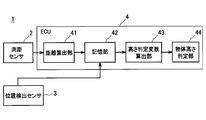•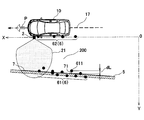••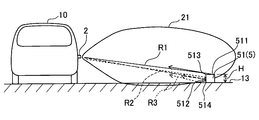••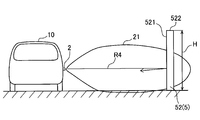••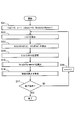•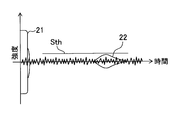•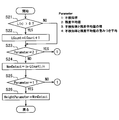•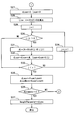•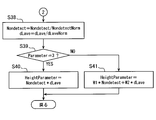•••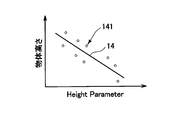•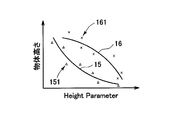•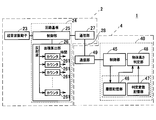••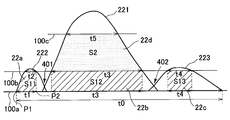•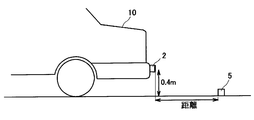•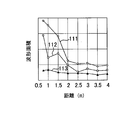•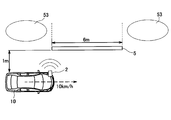•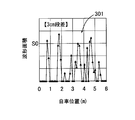•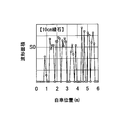••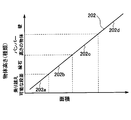•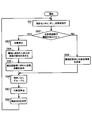••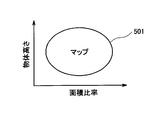•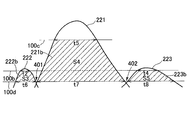••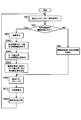••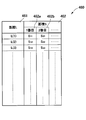Classifications

• GPHYSICS
• G01MEASURING; TESTING
• G01SRADIO DIRECTION-FINDING; RADIO NAVIGATION; DETERMINING DISTANCE OR VELOCITY BY USE OF RADIO WAVES; LOCATING OR PRESENCE-DETECTING BY USE OF THE REFLECTION OR RERADIATION OF RADIO WAVES; ANALOGOUS ARRANGEMENTS USING OTHER WAVES
• G01S7/00Details of systems according to groups G01S13/00, G01S15/00, G01S17/00
• G01S7/52Details of systems according to groups G01S13/00, G01S15/00, G01S17/00 of systems according to group G01S15/00
• G01S7/539Details of systems according to groups G01S13/00, G01S15/00, G01S17/00 of systems according to group G01S15/00 using analysis of echo signal for target characterisation; Target signature; Target cross-section
• GPHYSICS
• G01MEASURING; TESTING
• G01BMEASURING LENGTH, THICKNESS OR SIMILAR LINEAR DIMENSIONS; MEASURING ANGLES; MEASURING AREAS; MEASURING IRREGULARITIES OF SURFACES OR CONTOURS
• G01B11/00Measuring arrangements characterised by the use of optical means
• G01B11/02Measuring arrangements characterised by the use of optical means for measuring length, width or thickness
• G01B11/06Measuring arrangements characterised by the use of optical means for measuring length, width or thickness for measuring thickness, e.g. of sheet material
• GPHYSICS
• G01MEASURING; TESTING
• G01BMEASURING LENGTH, THICKNESS OR SIMILAR LINEAR DIMENSIONS; MEASURING ANGLES; MEASURING AREAS; MEASURING IRREGULARITIES OF SURFACES OR CONTOURS
• G01B7/00Measuring arrangements characterised by the use of electric or magnetic means
• G01B7/30Measuring arrangements characterised by the use of electric or magnetic means for measuring angles or tapers; for testing the alignment of axes
• GPHYSICS
• G01MEASURING; TESTING
• G01SRADIO DIRECTION-FINDING; RADIO NAVIGATION; DETERMINING DISTANCE OR VELOCITY BY USE OF RADIO WAVES; LOCATING OR PRESENCE-DETECTING BY USE OF THE REFLECTION OR RERADIATION OF RADIO WAVES; ANALOGOUS ARRANGEMENTS USING OTHER WAVES
• G01S15/00Systems using the reflection or reradiation of acoustic waves, e.g. sonar systems
• G01S15/88Sonar systems specially adapted for specific applications
• G01S15/93Sonar systems specially adapted for specific applications for anti-collision purposes
• G01S15/931Sonar systems specially adapted for specific applications for anti-collision purposes between land vehicles; between land vehicles and fixed obstacles
• GPHYSICS
• G01MEASURING; TESTING
• G01SRADIO DIRECTION-FINDING; RADIO NAVIGATION; DETERMINING DISTANCE OR VELOCITY BY USE OF RADIO WAVES; LOCATING OR PRESENCE-DETECTING BY USE OF THE REFLECTION OR RERADIATION OF RADIO WAVES; ANALOGOUS ARRANGEMENTS USING OTHER WAVES
• G01S13/00Systems using the reflection or reradiation of radio waves, e.g. radar systems; Analogous systems using reflection or reradiation of waves whose nature or wavelength is irrelevant or unspecified
• G01S13/88Radar or analogous systems specially adapted for specific applications
• G01S13/93Radar or analogous systems specially adapted for specific applications for anti-collision purposes
• G01S13/931Radar or analogous systems specially adapted for specific applications for anti-collision purposes between land vehicles; between land vehicles and fixed obstacles
• GPHYSICS
• G01MEASURING; TESTING
• G01SRADIO DIRECTION-FINDING; RADIO NAVIGATION; DETERMINING DISTANCE OR VELOCITY BY USE OF RADIO WAVES; LOCATING OR PRESENCE-DETECTING BY USE OF THE REFLECTION OR RERADIATION OF RADIO WAVES; ANALOGOUS ARRANGEMENTS USING OTHER WAVES
• G01S17/00Systems using the reflection or reradiation of electromagnetic waves other than radio waves, e.g. lidar systems
• G01S17/88Lidar systems specially adapted for specific applications
• G01S17/93Lidar systems specially adapted for specific applications for anti-collision purposes
• G01S17/936Lidar systems specially adapted for specific applications for anti-collision purposes between land vehicles; between land vehicles and fixed obstacles
• GPHYSICS
• G01MEASURING; TESTING
• G01SRADIO DIRECTION-FINDING; RADIO NAVIGATION; DETERMINING DISTANCE OR VELOCITY BY USE OF RADIO WAVES; LOCATING OR PRESENCE-DETECTING BY USE OF THE REFLECTION OR RERADIATION OF RADIO WAVES; ANALOGOUS ARRANGEMENTS USING OTHER WAVES
• G01S13/00Systems using the reflection or reradiation of radio waves, e.g. radar systems; Analogous systems using reflection or reradiation of waves whose nature or wavelength is irrelevant or unspecified
• G01S13/88Radar or analogous systems specially adapted for specific applications
• G01S13/93Radar or analogous systems specially adapted for specific applications for anti-collision purposes
• G01S13/931Radar or analogous systems specially adapted for specific applications for anti-collision purposes between land vehicles; between land vehicles and fixed obstacles
• G01S2013/9314Radar or analogous systems specially adapted for specific applications for anti-collision purposes between land vehicles; between land vehicles and fixed obstacles for parking operations
• GPHYSICS
• G01MEASURING; TESTING
• G01SRADIO DIRECTION-FINDING; RADIO NAVIGATION; DETERMINING DISTANCE OR VELOCITY BY USE OF RADIO WAVES; LOCATING OR PRESENCE-DETECTING BY USE OF THE REFLECTION OR RERADIATION OF RADIO WAVES; ANALOGOUS ARRANGEMENTS USING OTHER WAVES
• G01S13/00Systems using the reflection or reradiation of radio waves, e.g. radar systems; Analogous systems using reflection or reradiation of waves whose nature or wavelength is irrelevant or unspecified
• G01S13/88Radar or analogous systems specially adapted for specific applications
• G01S13/93Radar or analogous systems specially adapted for specific applications for anti-collision purposes
• G01S13/931Radar or analogous systems specially adapted for specific applications for anti-collision purposes between land vehicles; between land vehicles and fixed obstacles
• G01S2013/9371Sensor installation details
• G01S2013/9378Sensor installation details in the back of the vehicle
• GPHYSICS
• G01MEASURING; TESTING
• G01SRADIO DIRECTION-FINDING; RADIO NAVIGATION; DETERMINING DISTANCE OR VELOCITY BY USE OF RADIO WAVES; LOCATING OR PRESENCE-DETECTING BY USE OF THE REFLECTION OR RERADIATION OF RADIO WAVES; ANALOGOUS ARRANGEMENTS USING OTHER WAVES
• G01S13/00Systems using the reflection or reradiation of radio waves, e.g. radar systems; Analogous systems using reflection or reradiation of waves whose nature or wavelength is irrelevant or unspecified
• G01S13/88Radar or analogous systems specially adapted for specific applications
• G01S13/93Radar or analogous systems specially adapted for specific applications for anti-collision purposes
• G01S13/931Radar or analogous systems specially adapted for specific applications for anti-collision purposes between land vehicles; between land vehicles and fixed obstacles
• G01S2013/9371Sensor installation details
• G01S2013/9385Sensor installation details on the side(s) of the vehicle
• GPHYSICS
• G01MEASURING; TESTING
• G01SRADIO DIRECTION-FINDING; RADIO NAVIGATION; DETERMINING DISTANCE OR VELOCITY BY USE OF RADIO WAVES; LOCATING OR PRESENCE-DETECTING BY USE OF THE REFLECTION OR RERADIATION OF RADIO WAVES; ANALOGOUS ARRANGEMENTS USING OTHER WAVES
• G01S15/00Systems using the reflection or reradiation of acoustic waves, e.g. sonar systems
• G01S15/88Sonar systems specially adapted for specific applications
• G01S15/93Sonar systems specially adapted for specific applications for anti-collision purposes
• G01S15/931Sonar systems specially adapted for specific applications for anti-collision purposes between land vehicles; between land vehicles and fixed obstacles
• G01S2015/932Sonar systems specially adapted for specific applications for anti-collision purposes between land vehicles; between land vehicles and fixed obstacles for parking operations
• GPHYSICS
• G01MEASURING; TESTING
• G01SRADIO DIRECTION-FINDING; RADIO NAVIGATION; DETERMINING DISTANCE OR VELOCITY BY USE OF RADIO WAVES; LOCATING OR PRESENCE-DETECTING BY USE OF THE REFLECTION OR RERADIATION OF RADIO WAVES; ANALOGOUS ARRANGEMENTS USING OTHER WAVES
• G01S15/00Systems using the reflection or reradiation of acoustic waves, e.g. sonar systems
• G01S15/88Sonar systems specially adapted for specific applications
• G01S15/93Sonar systems specially adapted for specific applications for anti-collision purposes
• G01S15/931Sonar systems specially adapted for specific applications for anti-collision purposes between land vehicles; between land vehicles and fixed obstacles
• G01S2015/932Sonar systems specially adapted for specific applications for anti-collision purposes between land vehicles; between land vehicles and fixed obstacles for parking operations
• G01S2015/933Sonar systems specially adapted for specific applications for anti-collision purposes between land vehicles; between land vehicles and fixed obstacles for parking operations for measuring the dimensions of the parking space when driving past
• G01S2015/935Sonar systems specially adapted for specific applications for anti-collision purposes between land vehicles; between land vehicles and fixed obstacles for parking operations for measuring the dimensions of the parking space when driving past for measuring the contour, e.g. a trajectory of measurement points, representing the boundary of the parking space
• GPHYSICS
• G01MEASURING; TESTING
• G01SRADIO DIRECTION-FINDING; RADIO NAVIGATION; DETERMINING DISTANCE OR VELOCITY BY USE OF RADIO WAVES; LOCATING OR PRESENCE-DETECTING BY USE OF THE REFLECTION OR RERADIATION OF RADIO WAVES; ANALOGOUS ARRANGEMENTS USING OTHER WAVES
• G01S7/00Details of systems according to groups G01S13/00, G01S15/00, G01S17/00
• G01S7/02Details of systems according to groups G01S13/00, G01S15/00, G01S17/00 of systems according to group G01S13/00
• G01S7/41Details of systems according to groups G01S13/00, G01S15/00, G01S17/00 of systems according to group G01S13/00 using analysis of echo signal for target characterisation; Target signature; Target cross-section
• G01S7/411Identification of targets based on measurements of radar reflectivity

Abstract

Provided is an object detection device (1) mounted to a mobile body (10), said device comprising a transmission/reception unit (2, 23) which repeatedly transmits probing waves to the vicinity of the mobile body, and receives reflected waves which are reflected when the probing waves reach an object (5). A reception result calculation unit (41, 26) calculates as a detection distance the distance to the object on the basis of the reflected waves received by the transmission/reception unit, or calculates the area of the reflected waves. A history storage unit (42, 26) stores a history of a reception result which is the detection distance or the area. A determination unit (44, 48) determines the height of the object on the basis of the history of the reception result.

Description

Object detection device Cross-reference of related applications

This disclosure is based on Japanese Patent Application No. 2012-222549 filed on October 4, 2012, the contents of which are incorporated herein by reference.

The present disclosure relates to an object detection device that detects an object existing around a moving body.

Judging the height of objects around the vehicle is effective in various situations such as moving the vehicle to a parking space. For example, if it can be determined that an object in the back of a parking space for parallel parking is a wall, the vehicle door is prevented from touching the wall when getting on and off by automatically parking at a position with sufficient room for that wall. it can. For example, if it can be determined that an object in the back of the parking space is a curb, the vehicle door does not come into contact with the curb when getting on and off, so that the car can be parked at a marginal position with respect to the curb. For example, if the height of the step can be determined, it is useful for determining whether or not the vehicle can get over the step.

As a technique related to the determination of the height of an object, Patent Document 1 proposes an obstacle determination device that determines a low obstacle such as a ring-shaped ring and a high obstacle such as a pole or a wall. In the obstacle determination apparatus, the peak value of the reflected wave reflected when the exploration wave transmitted around the vehicle hits the detection target is detected. Then, when the peak value increases as the vehicle approaches the detection target, it is determined as a high object (such as a wall), and when the peak value decreases, it is determined as a low object (such as a ring ring).

However, since the technique of Patent Document 1 uses whether the peak value when the moving body (vehicle) approaches the object increases or decreases, the technique is used when the moving body passes the side path of the object. Thus, it cannot be applied when the distance fluctuation between the moving body and the object is small (that is, when the peak value fluctuation of the reflected wave is small).

In the technique of Patent Document 1, it is necessary to make the difference between the peak values “negative” as the moving object approaches the object for an object having a low height (that is, it is necessary to reduce the peak value). Therefore, it is necessary to set sensor specifications and mounting requirements so that it is difficult to detect objects with a low height at a short distance. Therefore, there is a possibility that an object cannot be detected at a short distance. Furthermore, in the technique of Patent Document 1, for example, since the difference in the peak value fluctuation of the reflected wave is small between the step of about 3 cm in height and the curb of about 10 cm in height, it is difficult to distinguish between the step and the curb. is there.

Japanese Unexamined Patent Publication No. 2010-197351 (corresponding to US2010 / 0220550A1)

This disclosure is intended to provide an object detection device that can determine the height of an object when the distance between the moving body and the object is small or the height of an object at a short distance with high accuracy.

An object detection apparatus according to an aspect of the present disclosure is an object detection apparatus that is mounted on a moving body and detects an object that exists around the moving body, and includes a transmission / reception unit, a reception result calculation unit, and a history storage unit And a determination unit. The transmitting / receiving unit repeatedly transmits an exploration wave around the moving body, and receives a reflected wave reflected by the exploration wave hitting the object. The reception result calculation unit calculates a distance to the object as a detection distance based on the reflected wave received by the transmission / reception unit, or calculates an area of the reflected wave. The history storage unit stores a history of reception results that are the detection distance or the area calculated by the reception result calculation unit. The determination unit determines the height of the object based on a history of the reception result.

Detected distance history and area history are in accordance with the object height even if the distance between the moving object and the object is small. Therefore, the object height when the distance fluctuation with the object is small can be determined with high accuracy. Moreover, since it is not necessary to set sensor specifications and mounting requirements so as to make it difficult to detect an object with a low height at a short distance as in Patent Document 1, it is possible to determine the height of an object at a short distance with high accuracy.

The above and other objects, configurations, and advantages of the present disclosure will become more apparent from the following detailed description with reference to the following drawings. In the drawing
FIG. 1 is a block diagram illustrating a configuration of the object detection device according to the first embodiment of the present disclosure. FIG. 2 is a diagram illustrating a scene in which the object detection device is detecting an object on the side of the vehicle. FIG. 3 is a waveform diagram of the reflected wave. FIG. 4 is a diagram schematically showing a path of a reflected wave from a low object. FIG. 5 is a diagram schematically showing a reflected wave from a low object. FIG. 6 is a diagram schematically showing a path of a reflected wave from a high object. FIG. 7 is a diagram schematically showing a reflected wave from a high object. FIG. 8 is a flowchart illustrating an object height determination process executed by the ECU according to the first embodiment. FIG. 9 is a waveform diagram when the intensity of the reflected wave is equal to or less than the threshold value Sth. FIG. 10 is a flowchart showing details of the process of S15 of FIG. FIG. 11 is a flowchart showing processing following S23: Yes or S25: No in FIG. FIG. 12 is a flowchart showing processing subsequent to S36: No in FIG. FIG. 13 is a flowchart illustrating an example of details of the processing in S16 of FIG. FIG. 14 is a flowchart showing another example of the details of the process of S16 of FIG. FIG. 15 is a diagram illustrating a straight line as the function Fun of the height determination variable with respect to the object height. FIG. 16 is a diagram showing a parabola as the function Fun of the height determination variable with respect to the object height. FIG. 17 is a block diagram illustrating a configuration of the object detection device according to the second to fifth embodiments of the present disclosure. FIG. 18 is a waveform diagram of a reflected wave for explaining a method of calculating the area of the reflected wave. FIG. 19 is an enlarged view of the XIX portion of FIG. 18, and is a diagram illustrating a specific example of a method for calculating the total sum of the areas of reflected waves. FIG. 20 is a diagram illustrating a first experimental condition for verifying that the object height correlates with the area of the reflected wave. FIG. 21 is a diagram showing the results of the first experiment. FIG. 22 is a diagram for explaining a second experimental condition for verifying that the object height correlates with the area of the reflected wave. FIG. 23A is a diagram showing a result of the second experiment in the case where the object has a step of 3 cm in height. FIG. 23B is a diagram showing a result of the second experiment in the case where the object is a curb with a height of 10 cm. FIG. 24 is a diagram illustrating the relationship between the area of the reflected wave and the value of the object height. FIG. 25 is a diagram illustrating the relationship between the area of the reflected wave and the type of object height. FIG. 26 is a flowchart of object height determination processing executed by the object detection device of the second embodiment. FIG. 27 is a conceptual diagram of an area and distance storage area stored in the history storage unit. FIG. 28 is a diagram showing the relationship between the area ratio between reflected waves and the object height. FIG. 29 is an enlarged view of the XIX section of FIG. 18, and is a diagram illustrating a specific example of a method for individually calculating the area of each reflected wave. FIG. 30 is a flowchart illustrating object height determination processing executed by the object detection device of the third embodiment. FIG. 31 is a flowchart illustrating object height determination processing executed by the object detection device of the fourth embodiment. FIG. 32 is a flowchart illustrating object height determination processing executed by the object detection device of the fifth embodiment. FIG. 33 is a conceptual diagram of an area and distance storage area stored in the history storage unit of the fifth embodiment.

(First embodiment)
Hereinafter, the object detection device 1 according to the first embodiment of the present disclosure will be described with reference to the drawings. In addition, this 1st Embodiment is embodiment of invention which determines object height based on the log | history of detection distance. FIG. 1 is a block diagram showing the configuration of the object detection device 1 of the present embodiment. The object detection device 1 is mounted on a vehicle 10 (see FIG. 2) as a moving body. The object detection device 1 includes a distance measuring sensor 2, a position detection sensor 3, and an ECU 4. The distance measuring sensor 2 is a sensor for detecting a distance to an object (a parked vehicle, a back wall of a parking space, a curb, etc.) existing on the side of the vehicle 10. The distance measuring sensor 2 is attached to the side surface (right side surface, left side surface) of the vehicle 10, for example. The distance measuring sensor 2 is attached to a bumper height of the vehicle 10, for example. The distance measuring sensor 2 repeatedly transmits a search wave 21 such as an ultrasonic wave (for example, a sound wave of 20 to 100 kHz) at a predetermined interval (for example, every several milliseconds) to the side of the vehicle 10 based on an instruction from the ECU 4.

FIG. 2 illustrates the transmission range of the exploration wave 21. The directivity of the transmission range is, for example, about 70 to 120 degrees. The center line of the transmission range (the front direction of the distance measuring sensor 2) is substantially parallel to the vehicle width direction (left-right direction) of the vehicle 10, for example. In addition, the center line may be inclined to, for example, about 20 degrees with respect to the vehicle width direction. The maximum detection distance at which the distance measuring sensor 2 can detect an object is, for example, about 4 m to 10 m. The distance measuring sensor 2 receives the reflected wave reflected by the transmitted exploration wave 21 hitting an object. Here, FIG. 3 shows waveforms of the exploration wave 21 and the reflected wave 22 with respect to time. The distance measuring sensor 2 notifies the ECU 4 of the timing (reception time) Tr when the intensity S (amplitude) of the received reflected wave 22 exceeds a predetermined threshold value Sth.

The distance measuring sensor 2 may be any sensor that transmits the exploration wave and receives the reflected wave, and may use a sound wave, a light wave, or a radio wave. good. As the distance measuring sensor 2, for example, a sensor such as an ultrasonic sensor, a laser radar, or a millimeter wave radar can be used.

The position detection sensor 3 is a sensor for detecting the position of the distance measuring sensor 2 (hereinafter referred to as sensor position) when the distance measuring sensor 2 detects the distance to the object. Specifically, the position detection sensor 3 includes a vehicle speed sensor that detects the vehicle speed, a steering angle sensor that detects the steering angle of the steering of the vehicle 10, and the like. The vehicle speed sensor is used to detect the distance traveled by the vehicle 10 (ranging sensor 2). The steering angle sensor is used to detect the traveling direction of the vehicle 10 (ranging sensor 2). The detection value of the position detection sensor 3 is input to the ECU 4.

The ECU 4 is mainly composed of a microcomputer composed of a CPU, a ROM, a RAM, and the like. The ECU 4 executes various processes such as determining the height of an object existing on the side of the vehicle 10 based on the detection value of the distance measuring sensor 2 and the detection value of the position detection sensor 3. As shown in FIG. 1, the ECU 4 includes a distance calculation unit 41, a storage unit 42, a height determination variable calculation unit 43, and an object height determination unit 44. The distance calculation unit 41, the height determination variable calculation unit 43, and the object height determination unit 44 may be physically separated from each other or may be functionally realized by a single microcomputer. Details of processing performed by the ECU 4 including the distance calculation unit 41, the height determination variable calculation unit 43, and the object height determination unit 44 will be described later. The storage unit 42 is a memory such as a RAM or a flash memory capable of storing various types of information.

Next, an object detection scene by the object detection device 1 will be described. FIG. 2 is a diagram illustrating an example of an object detection scene. Specifically, FIG. 2 shows a scene in which the distance to the object 5 is repeatedly detected and the height of the object 5 is detected while the vehicle 10 is moving along the side path 17 of the object 5 (upper side). Figure seen from). The object 5 is arranged along the side path 17, and is, for example, a curb or a wall arranged in the back of the parking space 200 (a space between the object 5 and the vehicle 10), or arranged in the parking space 200. The vehicle 10 may be a step that can be climbed over.

Here, FIGS. 4 to 7 are views of the vehicle 10 and the object 5 as viewed from the front side or the rear side of the vehicle 10, and are diagrams for explaining the difference in the reflected wave depending on the height of the object 5. FIG. FIG. 4 is a diagram schematically showing a path (reflection path) of a reflected wave when the object 5 is an object 51 (curbstone or the like) having a low height H. FIG. 5 is a diagram schematically showing reflected waves when the object 5 is an object 51 (curbstone or the like) having a low height H. As shown in FIG. 4, since the exploration wave 21 transmitted from the distance measuring sensor 2 hits a plurality of surfaces of the object 51, a plurality of reflection paths R exist. Specifically, the reflection path R1 when the exploration wave 21 hits the upper surface 511 of the object 51, the reflection path R2 when the exploration wave 21 hits the side face 512, the exploration wave 21 hits the side face 512, and then the ground 13 There is a reflection path R3. In addition to the reflection paths R1 to R3, for example, the reflection path (not shown) when the exploration wave 21 hits the boundary 513 between the upper surface 511 and the side surface 512, or the reflection path when hitting the boundary 514 between the side surface 512 and the ground (Not shown) is also possible.

As described above, since there are a plurality of reflection paths from the low object 51, the reflected waves of the respective reflection paths interfere with each other, and the reflected wave 220a is split into a plurality as shown in FIG. Therefore, the state of the reflected wave 220a varies greatly depending on the relative angle between the distance measuring sensor 2 and the object 51. Further, when the vehicle 10 is moving, the relative angle varies depending on the unevenness of the road 13 (ground). As shown in FIG. 5, for example, the distance measuring sensor 2 is horizontally oriented 2a, is directed upward 2b, is directed downward 2c, is directed forward or rearward of the vehicle 10 (not shown), etc. The direction of the distance measurement sensor 2 (relative angle with the object 51) fluctuates up and down, left and right when the vehicle 10 is moving. That is, when the vehicle 10 is moving, the relative angle between the distance measuring sensor 2 and the object 51 varies, and the reflected wave 220a from the object 51 varies greatly due to the variation. When the reflected wave 220a fluctuates, the distance to the object 51 cannot be detected, or even if the distance can be detected, the variation between the detected distances increases.

On the other hand, FIG. 6 is a diagram schematically showing a path (reflection path) of a reflected wave when the object 5 is an object 52 (wall or the like) having a high height H. FIG. 7 is a diagram schematically showing a reflected wave in the case of the high object 52. 6 and 7, the height H of the object 52 is higher than that of the vehicle 10. As shown in FIG. 6, in the case of a high object 52 such as a wall, most of the exploration wave 21 hits the side surface 521 of the object 52 and hardly hits other surfaces such as the upper surface 522. Therefore, the reflection path R of the exploration wave 21 can be considered as only the path R4 when it hits the side surface 521. Strictly speaking, there may be other reflection paths other than the reflection path R4, but the reflected waves from the other reflection paths are smaller in intensity than the reflected waves from the reflection path R4 and can be ignored. As a result, as shown in FIG. 7, the single reflected wave 220 b is received by the distance measuring sensor 2. Since the reflected wave 220b is single, it is more stable than the split reflected waves 220a in FIG. Therefore, when the vehicle 10 is moving, the orientation of the distance measuring sensor 2 changes such as the horizontally oriented state 2a, the upwardly oriented state 2b, and the downwardly oriented state 2c (the ranging sensor 2 and the object 52). Even if the relative angle of () changes), the reflected wave 220b is not affected so much. That is, the distance to the object 52 can be detected more stably when the object 52 is higher than when the object 51 is lower (see FIG. 5).

Therefore, the ECU 4 determines the height of the object from the history of detection distances repeatedly detected when the vehicle 10 is moving. The object height determination process executed by the ECU 4 will be described with reference to FIG. The process of FIG. 8 is started when the vehicle 10 starts traveling on a side route of the object, for example, in order to detect the object arranged in the back of the parking space. In the following description, the scene in FIG. 2 is assumed.

When the processing of FIG. 8 is started, first, the ECU 4 initializes each variable used in the following processing (S11). Specifically, the time t (n) = 0 when detecting the distance of the object 5 is set. In addition, the number of attempts to detect the distance of the object 5 (measurement count) n = 1 is set. In addition, the number of successful distance detections of the object 5 (the number of distance detections) LCount = 0 is set. Also, the normalization variable NondetectNorm = 1 is set. The meaning of the normalization variable NondetectNorm will be described later.

Next, the ECU 4 causes the distance measurement sensor 2 to transmit and receive the exploration wave and the reflected wave (S12). Thereafter, when the distance calculation unit 41 transmits the reception time Tr (see FIG. 3) of the reflected wave from the distance measuring sensor 2, the distance L (n) to the object 5 is transmitted based on the reception time Tr. ) Is calculated. Specifically, the distance calculation unit 41 calculates the detection distance L (n) based on the time T (see FIG. 3) between the transmission time Tt and the reception time Tr of the exploration wave and the sound speed. As shown in FIG. 9, when the intensity of the reflected wave 22 received by the distance measuring sensor 2 is equal to or smaller than the threshold value Sth, it is determined that the reflected wave could not be received and is sent from the distance measuring sensor 2 to the distance calculating unit 41. The reception time Tr is not transmitted. In this case, the distance calculation unit 41 sets the detection distance L (n) = 0, assuming that the distance cannot be detected. The distance calculation unit 41 may be provided in the distance measuring sensor 2.

Next, the ECU 4 detects the position of the distance measuring sensor 2 (with the measurement count n) (sensor n) when the current distance is detected based on the detection values (vehicle speed, steering angle) from the position detection sensor 3 (see FIG. 1). (Position) Attd is calculated (S13). Specifically, as shown in FIG. 2, for example, the position of the distance measuring sensor 2 at the time of starting the processing of FIG. 8 is the origin O, the traveling direction P of the vehicle 10 at that time is the X axis, and is perpendicular to the X axis. A coordinate system with the direction as the Y axis is set. The sensor position Attd is calculated as coordinates (AttdX (n), AttdY (n)) in the coordinate system. At this time, the moving distance of the vehicle 10 (ranging sensor 2) from the previous sensor position Attd can be calculated from the vehicle speed and time t (n). From the steering angle, the moving direction of the vehicle 10 (ranging sensor 2) from the previous sensor position Attd can be calculated. The current sensor position Attd is calculated from the moving distance and moving direction.

Next, the ECU 4 stores the detection distance L (n) calculated in S12 and the sensor position Attd calculated in S13 in the storage unit 42 (see FIG. 1) in association with the measurement count n (S14). In FIG. 2, a point separated by a detection distance L (n) in the Y-axis direction with reference to the sensor position Attd (AttdX (n), AttdY (n)) is illustrated as a distance detection point 6. The distance detection point 6 includes a point 61 when the distance can be detected and a point 62 when the distance cannot be detected (detection distance L (n) = 0). Thus, as the vehicle 10 moves on the side route 17, the sequence (history) of the distance detection points 61 can be set along the object 5 by repeatedly executing S 12 and S 13. On the other hand, as described above, when the vehicle 10 is moving, the relative angle between the distance measuring sensor 2 and the object 5 fluctuates. Therefore, depending on the relative angle, the distance cannot be detected (distance detection). There is also a point 62). In S14, it is synonymous with memorize | storing the state of the distance detection point 6 (distance detection point 61, 62) of FIG.

Next, the height determination variable calculation unit 43 (see FIG. 1) determines the height of the object 5 based on the history of the detection distance L (n) stored in the storage unit 42 (history of the distance detection point 6 in FIG. 2). A numerical value correlating with the height, that is, a height determination variable (Height Parameter) is calculated (S15). Here, FIG. 10 is a flowchart showing details of the processing of S15. Four examples will be described as height determination variables. The first example of the height determination variable is a non-detection rate that is a ratio of the number of times the distance cannot be detected with respect to the number of attempts to detect the distance to the object 5. As described above, when the height of an object such as a curb is low, the reflected wave is split. Therefore, the non-detection rate of a low object is higher than that of a high object. That is, the non-detection rate correlates with the height of the object 5.

The second example of the height determination variable is a residual average value. The residual average value is an average value of absolute values of residuals of the distance detection points 61 with respect to the approximate straight line 7 (see FIG. 2) of the history of the distance detection points 61. As described above, when the height of the object is low, the reflected wave is split, so that even if the distance can be detected, the variation between the distance detection points (detection distances) becomes large. That is, the residual average value of the low object is larger than the residual average value of the high object. That is, the residual average value correlates with the height of the object 5.

The third example of the height determination variable is the product of the non-detection rate and the residual average value. A fourth example of the height determination variable is a weighted average of the non-detection rate and the residual average value. The height determination variables in the third example and the fourth example use both the non-detection rate and the residual average value, and thus correlate with the height of the object 5.

10. Next to the flowchart of FIG. 10, a numerical value (Parameter) indicating which height determination variable is used in the first to fourth examples is illustrated. When the non-detection rate is set as the height determination variable, Parameter = 1, and when the residual average value is set as the height determination variable, Parameter = 2, and the product of the non-detection rate and the residual average value is set as the height determination variable. The processing of the flowcharts of FIG. 10 and the subsequent drawings will be described with Parameter = 3 when performing, and with Parameter = 4 when using the weighted average of the non-detection rate and the residual average value as the height determination variable.

10, the height determination variable calculation unit 43 first determines whether or not the detection distance L (n) at the current measurement count n is greater than zero (S21). That is, it is determined whether or not the distance can be detected. If the distance can be detected (L (n)> 0, S21: Yes), 1 is added to the distance detection count LCount (S22). After S22, the process proceeds to S23. If the distance cannot be detected in S21 (L (n) = 0, S21: No), the process proceeds to S23 without updating the distance detection count LCount in S22. In addition, whenever the measurement count n is updated in S18 of FIG. 8, S21 and S22 are repeatedly executed, so that the distance detection count LCount is incremented by 1 each time the distance is detected.

In S23, it is determined whether Parameter = 2. In other words, is the product of the non-detection rate of Parameter = 1, the non-detection rate of 3, 3 and the residual average value, the weighted average of the non-detection rate of 4 and the residual average value used as the height determination variable? It is determined whether the difference average value is used as a height determination variable (S23). The value of Parameter is set in advance in the ROM of the ECU 4 or the like, and it is only necessary to determine whether Parameter = 2 with reference to the set value.

If Parameter = 2 is not set, that is, if Parameter = 1, 3, or 4 (S23: No), the process proceeds to S24. In S24, the non-detection rate NonDect is calculated by substituting the measurement count n and the distance detection count LCount into Equation 1 below.

NonDect = (n-LCount) / n (Expression 1)
Next, it is determined whether or not Parameter = 1, that is, whether or not the non-detection rate is set as a height determination variable (S25). When Parameter = 1 (S25: Yes), the non-detection rate NonDetect calculated in S24 is set as a height determination variable (Height Parameter) (S26). Thereafter, the processing in FIG. 10 is terminated, and the process proceeds to S16 in FIG.

On the other hand, if Parameter = 2 in S23 (S23: Yes), or if Parameter = 1 is not satisfied in S25, that is, if Parameter = 3 or 4 (S25: No), the process proceeds to S27 in the flowchart of FIG. . The case of shifting to S27 corresponds to the case where any of the height determination variables of Parameter = 2, 3, and 4 is adopted.

In S27, each variable used in the subsequent processing is initialized. Specifically, the variable dLsum indicating the sum of the residuals dL of the distance detection points 61 with respect to the approximate straight line 7 in FIG. 2 is set to zero. Also, a variable Lsum indicating the sum of the detection distances of the measurement counts 1 to n is set to zero.

Next, based on the detection distance L (n) and the sensor position Attd stored in the storage unit 42, an approximate straight line Line = A × AttdX + B of the detection distance L (n) with respect to the sensor position Attd is calculated (S28). A and B are constants. In S28, the constants A and B are calculated using a method such as a least square method. That is, in S28, the approximate straight line 7 for the history of the distance detection point 61 in FIG. 2 is calculated. In calculating the approximate straight line Line, the point 62 when the distance cannot be detected is not used.

Next, the current measurement count j is set to 1 (S29). Next, it is determined whether or not the detection distance L (j) at the measurement count j is greater than zero, that is, whether or not the distance can be detected at the measurement count j (S30). When the detection distance L (j) is larger than zero, that is, when the distance can be detected (S30: Yes), the residual dL of the detection distance L (j) with respect to the approximate straight line calculated in S28 is calculated (S31). . That is, the residual dL = | A × AttdX (j) + B−L (j) | is calculated. The method of calculating the residual dL will be described using the example of the distance detection point 611 in FIG. 2. The X coordinate of the distance detection point 611 (the X coordinate of the sensor position Attd when the distance detection point 611 is calculated) Substituting AttdX), a point 71 on the approximate line 7 is obtained. The absolute value of the difference (Y coordinate difference) between the point 71 and the distance detection point 611 is calculated as the residual dL.

Next, the residual dL calculated in S31 is added to the total dLsum of the residual dL up to the previous time to update the total dLsum (S32). Further, the detection distance L (j) is added to the total Lsum of the detection distances up to the previous time, and the total Lsum is updated (S32). After S32, the process proceeds to S33.

On the other hand, if the detection distance L (j) is zero in S30, that is, if the distance cannot be detected with the measurement count j (S30: No), the processing of S30 to S32 is skipped and the process proceeds to S33.

In S33, it is determined whether or not the measurement count j has reached the latest measurement count n (S33). If not reached yet (S33: No), 1 is added to the measurement count j, and the measurement count j is updated to the next count value (S34). Thereafter, returning to S30, the above-described S30 to S33 are executed for the updated measurement count j. In this way, in S29 to S33, the residual dL with the approximate straight line 7 is calculated for each distance detection point 61 in FIG. 2, and the total dLsum of the residual dL and the total Lsum of each distance detection point 61 are calculated. is doing.

In S33, when the measurement count j reaches n (S33: Yes), the process proceeds to S35. In S35, the residual average value dLave is calculated by substituting the total dLsum of the residual dL and the distance detection count LCount into the following equation 2.

dLave = dLsum / Lcount (Formula 2)
In S35, the sum of the detection distances Lsum and the distance detection count LCount are substituted into Equation 3 below to calculate a normalization variable dLaveNorm for the residual average value. The normalization variable dLaveNorm is an average value of the detection distances, and a product of the non-detection rate and the residual average value or a weighted average value of the non-detection rate and the residual average value is used as the height determination variable. This is a variable for normalizing the residual average value.

dLaveNorm = Lsum / Lcount (Formula 3)
Next, it is determined whether Parameter = 2, that is, whether or not the residual average value is used as a height determination variable (S36). If Parameter = 2 (S36: Yes), the residual average value dLave calculated in S35 is set as a height determination variable (Height Parameter) (S37). Thereafter, the processing in FIG. 11 is terminated, and the process proceeds to S16 in FIG.

As described above, when the residual average value with respect to the approximate straight line of the detection distance history is used as the height determination variable, the traveling direction P of the vehicle 10 is inclined with respect to the object 5 as shown in FIG. Also, a highly accurate height determination variable can be obtained. When the variation in the detection distance (variance σ) is simply used as the height determination variable without using the approximate straight line, if the traveling direction of the vehicle is inclined with respect to the object, the variation in the detection distance becomes large. As a result, the variance σ becomes large.

On the other hand, if it is not Parameter = 2 in S36, that is, if Parameter = 3 or 4, the process proceeds to S38 in the flowchart of FIG. In S38, the non-detection rate Nondetect calculated in S24 of FIG. 10 is divided by the normalization variable NondetectNorm set in S11 of FIG. 8 to normalize the non-detection rate Nondetect. In this embodiment, since the normalization variable NondetectNorm = 1, the non-detection rate Nondetect calculated in S24 becomes the non-detection rate after normalization as it is. As a result, the non-detection rate Nondetect can be normalized as a variable between 0 and 1. Interpreting the meaning of the non-detection rate Nondetect after normalization, for example, that the non-detection rate Nondetect after normalization is zero means that the distance can be detected most (the number of times the distance cannot be detected is zero). A non-detection rate Nondetect after normalization of 1 means that the distance cannot be detected at all.

In S38, the residual average value dLave calculated in S35 of FIG. 11 is divided by the normalization variable dLaveNom calculated in S35 to normalize the residual average value dLave. As a result, the residual average value dLave can be normalized as a variable between 0 and 1. Interpreting the meaning of the normalized residual average value dLave means that the normalized residual average value dLave is zero means that the error (variation) between the residuals dL is the smallest. The average residual value dLave after normalization of 1 means that the error (variation) between the residuals dL is the largest (the same error as the detection distance occurs).

In this embodiment, the normalization variables NondetectNorm = 1 and dLaveNorm = Lsum / Lcount are used to normalize the non-detection rate and the residual average value. However, this is only an example, and the non-detection rate and the residual are normalized. If the average value can be converted into the same dimension, normalization may be performed by another method. For example, normalization variables NondetectNorm and dLaveNorm may be set in advance from experimental values and stored.

Next, whether Parameter = 3 or 4, that is, whether the product of the non-detection rate and the residual average value is the height determination variable, or the weighted average of the non-detection rate and the residual average value is the height determination variable Is determined (S39). When Parameter = 3 (S39: Yes), the product of the normalized non-detection rate Nondetect calculated in S38 and the normalized residual average value dLave is calculated as a height determination variable (Height Parameter). (S40). Thereafter, the processing in FIG. 12 is terminated, and the process proceeds to S16 in FIG. Thus, by using the product of the non-detection rate and the residual average value as the height determination variable, it is possible to obtain a height determination variable with higher accuracy than when either one is used as the height determination variable. it can.

In S39, if Parameter = 4 (S39: No), the weighted average of the non-detection rate Nondetect after normalization calculated in S38 and the residual average value dLave after normalization is calculated as a height determination variable (Height). Parameter) is calculated (S41). That is, when the weight of the non-detection rate is W1 and the weight of the residual average value is W2, HeightＨParameter = W1 × Nondetect + W2 × dLave is calculated (S41). The weights W1 and W2 are set in advance in consideration of which of the non-detection rate and the residual average value is higher when determining the object height. For example, if the reliability of the non-detection rate is higher than the reliability of the residual average value, W1> W2 is set. After S41, the process of FIG. 12 is terminated, and the process proceeds to S16 of FIG. As described above, by using the weighted average of the non-detection rate and the residual average value as the height determination variable, it is possible to obtain a height determination variable with higher accuracy than when either one is used as the height determination variable. be able to.

Returning to the description of FIG. 8, after calculating the height determination variable (HeightＨParameter) in S15, the process proceeds to S16. In S16, the object height determination unit 44 (see FIG. 1) determines the height of the object 5 (see FIG. 2) based on the height determination variable (Height Parameter) calculated in S15. Here, FIGS. 13 and 14 are flowcharts showing the details of the processing of S16. In S16, either the process of FIG. 13 or the process of FIG. 14 is executed. First, the processing of FIG. 13 will be described. A low object height determination variable (non-detection rate, residual average value or product thereof, weighted average) is larger than a high object height determination variable. Therefore, when the processing shifts to the processing in FIG. 13, first, it is determined whether or not the height determination variable (Height Parameter) is smaller than a preset threshold value Hth (S51). This threshold value Hth is a value of a height determination variable that classifies whether the height of the object is high or low. In the storage unit 42 (see FIG. 1), as the threshold Hth, the threshold Hth1 when the non-detection rate is the height determination variable, the threshold Hth2 when the residual average value is the height determination variable, and the non-detection rate And a threshold value Hth3 when the product of the residual average value is the height determination variable, and a threshold value Hth4 when the weighted average of the non-detection rate and the residual average value is the height determination variable. In S51, the threshold value Hth corresponding to the type of the height determination variable to be used is read and compared with the height determination variable.

If the height determination variable (Height Parameter) is smaller than the threshold value Hth (S51: Yes), it is determined that the object 5 is a high object such as a wall (S52). Thereafter, the process of FIG. 13 is terminated. On the other hand, when the height determination variable (Height Parameter) is larger than the threshold value Hth (S51: No), it is determined that the object 5 is a low object such as a curb (S53). Thereafter, the process of FIG. 13 is terminated. Thus, by using the threshold value Hth, the height of the object 5 can be easily determined.

Next, the process of FIG. 14 will be described. Explaining the premise of the processing in FIG. 14, it is considered that there is a correlation between the height determination variable (Height Parameter) and the object height value. Specifically, the height determination variable is considered to increase as the object height decreases. Therefore, an experiment is performed in advance to see how the height determination variable changes for objects of various heights. Then, a function of a height determination variable with respect to the object height is calculated based on the experimental result. Specifically, for example, as shown in FIG. 15, in the figure of the object height with respect to the height determination variable, an approximate straight line 14 of each point 141 obtained by experiment is calculated in advance. The approximate straight line 14 is stored in advance in the storage unit 42 as a function Fun (HeightParameter) = C × HeightParameter + D (C and D are constants) of the height determination variable with respect to the object height. As described above, when a straight line is adopted as the function Fun, the amount of calculation for calculating the object height can be reduced as compared with the case where a parabola described below is adopted.

In addition, as shown in FIG. 16, when the points obtained in the experiment are distributed like the points denoted by reference numeral 151, the downwardly convex parabola 15 is used as the function Fun of the height determination variable with respect to the object height. May be adopted. Further, when the points obtained in the experiment are distributed as indicated by the reference numeral 161, an upwardly convex parabola 16 may be employed as the function Fun of the height determination variable with respect to the object height. In this case, the parabolas 15 and 16 are stored in advance in the storage unit 42 as a function of the height determination variable Fun (HeightParameter) = E × HeightParameter 2 + F × HeightParameter + G (E, F, and G are constants) with respect to the object height. deep. Thus, when the parabola is adopted as the function Fun, the accuracy of the object height can be improved as compared with the case where the linear function Fun is adopted. It should be noted that a function other than a straight line or a parabola (quadratic curve), for example, an exponential function or a cubic or higher-order curve may be adopted as the function Fun according to the distribution state of the experimental points.

Note that a function Fun corresponding to the type of height determination variable to be used is prepared. That is, when the non-detection rate is set as the height determination variable, a function Fun of the non-detection rate with respect to the object height is obtained in advance. When the residual average value is used as a height determination variable, a function Fun of the residual average value with respect to the object height is obtained in advance. Further, when the product of the non-detection rate and the residual average value is used as a height determination variable, a function Fun of the product of the non-detection rate and the residual average value with respect to the object height is obtained in advance. When the weighted average of the non-detection rate and the residual average value is used as the height determination variable, a function of the weighted average of the non-detection rate and the residual average value with respect to the object height is obtained in advance.

For convenience of explanation in FIG. 14, a variable Method indicating the type of the function Fun stored in the storage unit 42 is defined. Method = 1 indicates that the function Fun is a straight line. Method = 2 indicates that the function Fun is a parabola. Method ≠ 1, 2 indicates that the function Fun is a function other than a straight line and a parabola.

Based on the above, in the process of FIG. 14, it is first determined whether Method = 1 (S54). If Method = 1 (S54: Yes), a straight line (C × HeightParameter + D) as a function Fun is read from the storage unit 42 (S55). Thereafter, the process proceeds to S59.

In S54, when Method = 1 is not satisfied (S54: No), the process proceeds to S56 to determine whether Method = 2. In the case of Method = 2 (S56: Yes), a parabola (E × HeightParameter2 + F × HeightParameter + G) as a function Fun is read from the storage unit 42 (S57). Thereafter, the process proceeds to S59.

If it is not Method = 2 in S56 (S56: No), the process proceeds to S58, and other functions (exponential function, cubic curve function, etc.) are read from the storage unit 42. Thereafter, the process proceeds to S59.

In S59, the height Heigt of the object 5 is calculated by substituting the value of the height determination variable calculated in S15 of FIG. 8 into the function Fun read in S55, S57, or S58 (S59). Thereafter, the processing in FIG. 14 is terminated.

Returning to the description of FIG. 8, after calculating the height of the object 5 in S16, the process proceeds to S17. In S17, it is determined whether or not the process of FIG. 8 is to be ended by determining whether or not a predetermined end condition is satisfied. Specifically, for example, when the parking space 200 of FIG. 2 can be detected, it is determined that a predetermined end condition is satisfied. Further, when the process of FIG. 8 cannot be continued, for example, when an obstacle approaches the vehicle 10, it is determined that a predetermined end condition is satisfied. If the end condition is not satisfied (S17: No), the process proceeds to S18, and the measurement count n is updated to the next value (n = n + 1) (S18). Thereafter, the process returns to S12, and the processes of S12 to S17 described above are executed for the latest measurement count n. As described above, until the end condition is satisfied in S17, the processing of S12 to S17 is repeated, and as a result, the number of detected distance data stored in the storage unit 42 gradually increases. As the number of data of the detection distance increases, the accuracy of the height determination variable is improved, and as a result, the accuracy of the object height is improved.

If the end condition is satisfied in S17 (S17: Yes), the process of FIG. Thereafter, the ECU 4 executes a process according to the height of the object 5 determined in S16. For example, when the vehicle 10 is automatically parked in the detected parking space 200, if the object 5 is a high object such as a wall, the ECU 4 parks the vehicle 10 at a position where there is room for the object 5. . Thereby, it can prevent that a vehicle door contacts the object 5 (wall) at the time of getting on / off. For example, when the object 5 is a low object such as a curbstone, the vehicle 10 is parked at a marginal position with respect to the object 5. Thereby, it can prevent that the vehicle 10 protrudes from the parking space 200. FIG. Further, when the height value of the object 5 is calculated in the process of FIG. 14, whether or not the vehicle 10 can get over the object 5 based on the value, and whether or not the object 5 may come into contact with the bumper. Etc. may be determined.

As described above, in this embodiment, paying attention to the fact that the fluctuation of the reflected wave due to the relative angle fluctuation between the object and the distance measuring sensor differs depending on the height of the object, the variable reflecting the fluctuation of the reflected wave ( The object height is determined based on a non-detection rate, a residual average value, or a product thereof, a weighted average). Therefore, the object height can be accurately determined even when the distance between the vehicle and the object is small, such as when the vehicle is moving along the side path of the object. In the above embodiment, four examples have been described as the height determination variable. However, for example, the sum of the non-detection rate and the residual average value may be used as the height determination variable. This also makes it possible to obtain a height determination variable that reflects both the non-detection rate and the residual average value, thereby improving the accuracy of the object height.
(Second Embodiment)
Next, the object detection device 1 according to the second embodiment of the present disclosure will be described. The second embodiment is an embodiment of the invention for determining the object height based on the area history of the reflected wave.
FIG. 17 is a block diagram illustrating a configuration of the object detection device 1 of the present embodiment. The object detection device 1 is mounted on a vehicle 10 (see FIGS. 20 and 22). The object detection device 1 includes a distance measuring sensor 2 and an ECU 4. The distance measuring sensor 2 is an example of a sensor unit, and the ECU 4 is an example of a control unit.

The distance measuring sensor 2 is a sensor for detecting an object existing around the vehicle 10 (back, front, side, etc.). In this embodiment, an example in which an ultrasonic sensor is employed as the distance measuring sensor 2 will be described. However, if the distance measuring sensor 2 is a sensor that transmits an exploration wave and receives a reflected wave, another type of sensor is used. It may be adopted. The distance measuring sensor 2 is attached to, for example, the height positions of bumpers on the rear surface, front surface, and side surfaces of the vehicle 10. As shown in FIG. 1, the distance measuring sensor 2 is configured to accommodate the ultrasonic transducer 23, the circuit board 24, and the communication unit 27 in the same casing. The ultrasonic vibrator 23 is a piezoelectric vibrator that transmits and receives ultrasonic waves using the piezoelectric effect. That is, when a drive signal is given to the ultrasonic transducer 23, the ultrasonic transducer 23 vibrates, and the ultrasonic wave as the exploration wave is transmitted to the outside (rear, front, side, etc. of the vehicle 10) by the vibration. . Then, when receiving the reflected wave (ultrasonic wave) reflected by the transmitted exploration wave hitting the object, the ultrasonic transducer 23 generates an electrical signal corresponding to the reflected wave by the piezoelectric effect. The electric signal (reflected wave) is input to the control unit 25 described later.

The circuit board 24 is a board on which a control unit 25 and an area calculation unit 26 are mounted as processing circuits that execute processing related to transmission of exploration waves and reception of reflected waves. The control unit 25 is connected to the ultrasonic transducer 23, generates a drive signal for driving (vibrating) the ultrasonic transducer 23 based on an instruction from the ECU 4, and uses the drive signal as the ultrasonic transducer. 23. And the control part 25 makes the ultrasonic transducer | vibrator 23 transmit a search wave repeatedly at predetermined intervals (for example, every 100 milliseconds). In addition, the control unit 25 has a timing (reception time) when the amplitude (intensity) of the reflected wave input from the ultrasonic transducer 23 exceeds a predetermined threshold (distance calculation threshold for calculating the distance to the object). Is notified to the ECU 4. In addition, the control unit 25 inputs the reflected wave input from the ultrasonic transducer 23 to the area calculation unit 26.

The area calculation unit 26 is a part that calculates the area of the reflected wave input from the control unit 25. Here, FIG. 18 illustrates the waveforms of the exploration wave 21 and the reflected wave 22 with respect to time. FIG. 18 shows the waveforms of the reflected waves 221, 222, and 223 divided into three. In the waveform diagram of FIG. 18, the area calculating unit 26 sets a plurality of threshold lines 100 of the amplitude of the reflected wave, and calculates the areas of the portions 221a to 223a of the reflected waves 221 to 223 surrounded by the lines 100. is doing. Specifically, the area calculation unit 26 includes a plurality of counters 261 (see FIG. 17). Each counter 261 corresponds to one of the lines 100 (threshold values) in FIG. 18 and receives a reflected wave input from the control unit 25. The counter 261 then counts the time during which the amplitude of the input reflected wave exceeds the threshold value assigned to itself (threshold time), and outputs the threshold time.

The area calculation unit 26 calculates the total sum of the trapezoidal areas surrounded by the threshold time output from each counter 261. Here, with reference to FIG. 19, a specific example of a method for calculating the total sum of areas from the threshold time excess will be described. FIG. 19 is an enlarged view of the XIX portion of FIG. FIG. 19 shows the waveforms of the reflected waves 221, 222, and 223 that are split into three. The reflected wave 222 shown on the left is received first, the reflected wave 221 shown in the middle is received second, and the right It shows that the illustrated reflected wave 223 has been received last. The reflected waves 221 to 223 partially overlap each other. Note that. The amplitude of the second reflected wave 221 is the largest. In the example of FIG. 19, the first reflected wave 222 and the third reflected wave 223 have the same height (amplitude). In the example of FIG. 19, the branch point 401 of the first reflected wave 222 and the second reflected wave 221 and the branch point 402 of the second reflected wave 221 and the third reflected wave 223 have the same height. It has become.

Further, FIG. 19 shows the minimum threshold line 100a, the threshold line 100b set near the peak of the first reflected wave 222 and the peak of the third reflected wave 223, and the second reflected wave 221. A threshold line 100c set in the vicinity of the peak is shown. Note that the branch points 401 and 402 appear in the vicinity of the line 100a (slightly above the line 100a).

The time t0 is output from the counter 261 corresponding to the line 100a. The time t0 includes the width near the root of the first reflected wave 222 (strictly, the portion 222a in FIG. 18) and the width near the root of the second reflected wave 221 (strictly, the portion 221a in FIG. 18). This corresponds to the total width of the third reflected wave 223 (strictly speaking, the portion 223a in FIG. 18) near the base.

From the counter 261 corresponding to the line 100b, the time t2 is output, and once the output is stopped, the time counting is restarted immediately and the time t3 is output. After the output is once again stopped, the time counting is immediately started. Resume and time t4 is output. The time t2 corresponds to the width near the peak of the first reflected wave 222. The time t3 corresponds to the width near the middle of the second reflected wave 221. The time t4 corresponds to the width near the peak of the third reflected wave 223.

The time t5 is output from the counter 261 corresponding to the line 100c. The time t5 corresponds to the width near the peak of the second reflected wave 221.

The area calculation unit 26 calculates the area surrounded by the line 100a and the line 100b, but the line 100b (time t2, t3, t4) is broken by the reflected waves 221 to 223, whereas the line 100a (time Since no break occurs at t0), it is difficult to set a trapezoidal portion. Therefore, in this case, for example, the trapezoidal part (or rectangular part) is set as follows. Specifically, the area calculation unit 26 finishes counting the threshold time exceeding the threshold value of the first reflected wave 222 when the counter 261 of the line 100a starts counting the threshold value time and the counter 261 of the line 100b. A time t1 between the time points P2 is calculated. Then, the area calculation unit 26 calculates the area S11 of the trapezoidal portion 22a with the time t1 as the lower side, the time t2 as the upper side, and the height between the lines 100a and 100b. The area calculation unit 26 projects the time t3 on the line 100b onto the line 100a, the time t3 on the projected line 100a is the lower side, the time t3 on the line 100b is the upper side, and between the lines 100a and 100b. An area S12 of a trapezoidal portion (in this case, a rectangular portion) 22b having a height is calculated. The area calculation unit 26 projects the time t4 on the line 100b onto the line 100a, the time t4 on the projected line 100a is the lower side, the time t4 on the line 100b is the upper side, and between the lines 100a and 100b. The area S13 of the trapezoidal portion (in this case, the rectangular portion) 22c having the height is calculated. The area calculation unit 26 sets these areas S11, S12, and S13 as an area surrounded by the line 100a and the line 100b (hatched hatched portion).

The area calculation unit 26 calculates the area S2 of the trapezoidal portion 22d (dot hatched portion) where the time t3 is the lower side, the time t5 is the upper side, and the height between the lines 100b and 100c is the area surrounded by the lines 100b and 100c. calculate. Then, the area calculation unit 26 adds the calculated areas S11, S12, S13, and S2. The area after the addition corresponds to the sum of the areas of the portions 221a, 222a, and 223a in FIG.

When the area calculation method described in FIG. 19 is generalized, the time from the counter 261 corresponding to the minimum threshold among the times output from the plurality of counters 261, and the threshold set near the branch point of the reflected wave are set. The trapezoidal portion is set by selecting the time from the corresponding counter 261 and the time from the counter 261 corresponding to the threshold set near the peak of the reflected wave. And the area of each set trapezoid part is calculated, and each calculated area is added. As a result, even if a plurality of reflected waves partially overlap, the sum of the areas of the reflected waves can be calculated. Note that the area calculation method described with reference to FIG. 19 is merely an example, and the total sum of the areas of the reflected waves can be calculated by appropriately setting the trapezoidal part based on the threshold time exceeding each counter 261.

Returning to the description of FIG. 17, the area calculation unit 26 transmits the calculated reflected wave area to the control unit 25. The control unit 25 transmits the area to the ECU 4.

The communication unit 27 is connected to the communication unit 49 of the ECU 4 via a wire harness (communication line) 28, and is a part that transmits and receives various data between the control unit 25 and the ECU 4. Specifically, for example, the communication unit 27 transmits various data from the control unit 25 (the timing (reception time when the reflected wave exceeds the distance calculation threshold, the area of the reflected wave) to the ECU 4 as a digital value. Further, the communication unit 27 receives various data (such as a search wave transmission instruction signal) from the ECU 4 and transmits the received data to the control unit 25.

The ECU 4 is mainly composed of a microcomputer composed of a CPU, a ROM, a RAM, and the like. The ECU 4 is arranged at a position different from the distance measuring sensor 2 (for example, the back side of the instrument panel). The ECU 4 includes a processing circuit 40 that performs various processes for determining the height of an object existing around the vehicle 10, and a communication unit 49 connected to the processing circuit 40 (control unit 45). The processing circuit 40 includes a control unit 45, a history storage unit 46, a determination variable storage unit 47, and an object height determination unit 48. The control unit 45 instructs the ranging sensor 2 to transmit an exploration wave, calculates the distance to the object based on the reception time of the reflected wave from the ranging sensor 2, and the like around the vehicle 10 Various processes for detecting an object are performed. The object height determination unit 48 performs various processes for determining the height of the detected object. Details of processing performed by the control unit 45 and the object height determination unit 48 will be described later.

The history storage unit 46 and the determination variable storage unit 47 are memories such as a RAM, a ROM, and a flash memory that can store various data. The history storage unit 46 stores the history of the area calculated by the distance measuring sensor 2 (area calculation unit 26). The determination variable storage unit 47 stores a map indicating the relationship of the object height to the reflected wave area for determining the height of the object. Details of the map will be described later.

The communication unit 49 is connected to the control unit 45 and is a part that transmits and receives various data between the control unit 45 and the distance measuring sensor 2. Specifically, for example, the communication unit 49 receives the area of the reflected wave from the distance measuring sensor 2 and transmits the received area to the control unit 45.

Next, knowledge that is a premise of the object height determination method in the present embodiment will be described. The total energy of the reflected wave received by the distance measuring sensor 2 (the sum of the energy of each reflected wave when the reflected wave is split into a plurality) varies depending on the height of the object hit by the exploration wave. Specifically, when the exploration wave hits a high object such as a wall, most of the exploration wave hits the object, so the total energy of the reflected wave increases. On the other hand, when the exploration wave hits a low object such as a curb or a step, a part of the exploration wave does not hit the object and passes behind the object, so that the total energy of the reflected wave becomes small.

The present inventors conducted two experiments to verify that the object height correlates with the area of the reflected wave. FIG. 20 is a diagram for explaining one experimental condition of the two experiments. As a first experimental condition, as shown in FIG. 20, the distance measuring sensor 2 is installed at a height (0.4 m) corresponding to the height of the bumper of the vehicle 10, and is located at a position facing the distance measuring sensor 2. The object 5 is installed. The waveform area of the reflected wave when the distance between the distance measuring sensor 2 and the object 5 is changed is measured. At this time, the object 5 is a wall, a curb with a height of 10 cm, or a step with a height of 3 cm, and the waveform area of the reflected wave is measured for each of the wall, the curb, and the step. Note that the experimental conditions in FIG. 20 assume a scene in which the object 5 behind or in front of the vehicle 10 is detected when the vehicle 10 is backing or moving forward during a parking operation or the like.

FIG. 21 shows the experimental results. The horizontal axis in FIG. 21 indicates the distance between the distance measuring sensor 2 and the object 5, and the vertical axis in FIG. 21 indicates the waveform area. A line denoted by reference numeral 111 indicates an experimental result when the object 5 is a wall. A line denoted by reference numeral 112 indicates an experimental result when the object 5 is a curbstone having a height of 10 cm. A line denoted by reference numeral 113 indicates an experimental result when the object 5 has a height difference of 3 cm. Note that each plot point 114 constituting each line 111 to 113 is an average of N = 300 waveform areas.

21. From FIG. 21, at any distance, the higher the object, the larger the waveform area. That is, line 111 (wall)> line 112 (10 cm curb)> line 113 (3 cm step). Even if the object is the same, the waveform area changes as the distance changes. Specifically, for a particularly high object (wall), the waveform area increases as the distance decreases.

FIG. 22 is a diagram for explaining the second experimental condition. As a second experiment condition, an object 5 having a length of 6 m is installed as shown in FIG. The object 5 is a 3 cm high step or a 10 cm high curb. The vehicle 5 is moved at a speed of 10 km per hour along the side path of the object 5 with a distance of 1 m from the object 5. At this time, the exploration wave is repeatedly transmitted to the side of the vehicle 10 (toward the object 5), and the waveform area of the reflected wave of the exploration wave is measured. Note that the experimental conditions in FIG. 22 assume, for example, a scene in which the vehicle 10 is moving along a side path of the parking space in order to detect a curb or a step disposed in the parking space.

FIG. 23A shows the experimental results when the object 5 is a step with a height of 3 cm, and FIG. 23B shows the experimental results when the object 5 is a curb with a height of 10 cm. The horizontal axis of FIG. 23A and FIG. 23B has shown the position in the length direction of the object 5 of the vehicle 10. FIG. The vertical axis of FIG. 23A and FIG. 23B indicates the waveform area of the reflected wave. As shown in FIG. 23A and FIG. 23B, it can be seen that the measured waveform area varies in both the step of 3 cm height and the curbstone of 10 cm height. This is because when the vehicle 10 is moving, the relative angle between the distance measuring sensor 2 and the object 5 varies, and the reflected wave varies due to the variation. It also depends on the instability of the exploration wave (ultrasonic wave) itself transmitted from the distance measuring sensor 2.

Also, comparing FIG. 23A and FIG. 23B, FIG. 23B has more times than a certain waveform area S0 than FIG. 23A. That is, although the waveform area varies, the overall area history shows that the higher the object, the larger the waveform area.

From the above, as shown in FIGS. 24 and 25, a map can be set in which the object height increases as the area of the reflected wave increases. FIG. 24 shows a relationship (map) 201 between the reflected wave area and the object height value. FIG. 25 shows a relationship (map) 202 between the area of the reflected wave and the object height. In the map 202 of FIG. 25, as an example, in the region 202a where the reflected wave area is the smallest, the object height (object type) is set to an object height (about 3 cm) that the vehicle can get over, and the reflected wave area is the next. In the small area 202b, the object height is set to the curb height (about 10 cm), and in the area 202c next to the area 202b, the object height is set to an object height (about 40 cm) that may come into contact with the bumper of the vehicle. In the above region 202d, the object height is the height of the wall. 24 and 25 show maps 201 and 202 in which the object height changes approximately in proportion to the area of the reflected wave for convenience of explanation, the object height is the reflected wave. It is not necessarily proportional to the area.

In the present embodiment, the maps 201 and 202 of FIGS. 24 and 25 are obtained in advance by experiments, and one or both of the maps 201 and 202 are stored in advance in the determination variable storage unit 47 (see FIG. 17). ing.

Next, processing when the object detection device 1 of the present embodiment determines the object height will be described. FIG. 26 is a flowchart showing the processing. In FIG. 26, the process which ECU4, the control part 25 of the ranging sensor 2, and the area calculation part 26 perform is shown as one flowchart. The process of FIG. 26 is performed when a predetermined condition for starting detection of an object is satisfied (for example, when traveling on a side path of the parking space is started in order to detect the parking space, or a parking operation for the parking space is started. To start). And it complete | finishes when the predetermined conditions which complete | finish the detection of an object are satisfy | filled (for example, when parking space was detected or parking to parking space was completed).

26 is started, first, the ECU 4 (control unit 45) instructs the distance measurement sensor 2 to transmit an exploration wave at predetermined intervals (S61). Receiving the transmission instruction, the control unit 25 of the distance measuring sensor 2 causes the ultrasonic transducer 23 to transmit exploration waves at predetermined intervals (S61). Thereafter, when the amplitude of the reflected wave input from the ultrasonic transducer 23 exceeds the threshold (distance calculation threshold), the control unit 25 notifies the ECU 4 of the exceeded timing (reception time) (S61). . The control unit 25 does not notify the ECU 4 of the reception time when the amplitude of the reflected wave does not exceed the threshold value (distance calculation threshold value).

Next, the control unit 45 of the ECU 4 determines whether or not the amplitude of the reflected wave exceeds the distance calculation threshold based on whether or not the reception time is notified from the distance measuring sensor 2 (S62). When the reception time is notified (when the amplitude of the reflected wave exceeds the distance calculation threshold) (S62: Yes), the process proceeds to S63. In S63, the control unit 45 of the ECU 4 calculates the distance to the object by multiplying the time T between the exploration wave transmission time Tt and the reflected wave reception time Tr by the speed of sound, as shown in FIG. S63).

Next, as described with reference to FIGS. 18 and 19, if there is a portion of the reflected wave surrounded by the threshold time exceeding the counter 261, the area calculation unit 26 calculates the total area of the portions. (S64). The control unit 25 transmits the area to the ECU 4 (S64).

Next, the control unit 45 of the ECU 4 stores the area calculated in S64 in the history storage unit 46 (see FIG. 17) in association with the distance calculated in S63 (S65). Here, FIG. 27 shows a conceptual diagram of the area and distance storage area 460 stored in the history storage unit 46. The storage area 460 is provided with a distance storage area 461 for storing the distance at each measurement count and an area storage area 462 for storing the area S of the reflected wave at each measurement count. Each column of the area storage area 462 is associated with each column of the distance storage area 461. The numbers in parentheses of the distance L and the area S stored in the storage areas 461 and 462 indicate the value of the measurement count. The storage areas 461 and 462 store distances and areas in the order of measurement counts, that is, in the order in which distances and areas are measured.

On the other hand, in S62, when there is no notification of the reception time from the distance measuring sensor 2 (when the amplitude of the reflected wave does not exceed the threshold for distance calculation) (S62: No), the process proceeds to S69. In this case, the area calculation by the area calculation unit 26 is not performed. In S69, the control unit 45 adds undetected information (distance L = 0, area S = 0) to the storage region (see FIG. 27) corresponding to the current measurement count in the history storage unit 46 (S69). When the horizontal axis in FIG. 23 is regarded as the measurement count, the area history stored in the history storage unit 46 is graphed as shown in FIG.

After S65 or after S69, the process proceeds to S66, where the object height determination unit 48 refers to the distance history stored in the history storage unit 46, and displays the area history stored in the history storage unit 46 for each object. (S66). The purpose of the process of S66 will be described with reference to FIG. Assume that the height of the object 5 in FIG. 22 is being determined. At this time, in the process of calculating the area of the reflected wave from the object 5 while the vehicle 10 moves along the side path of the object 5 and storing the area history, the reflection from the object 53 different from the object 5 is performed. The area of the wave may be added to the area history. When the area history of the reflected wave of the object 5 is stored, the distance history corresponding to the object 5 is stored in association with the area history. When the area history of the reflected wave of another object 53 is stored, a distance history corresponding to the object 53 is stored in association with the area history. Therefore, by viewing the distance history, the area history of the object 5 and the area history of another object 53 can be separated.

Referring to FIG. 27 as an example, the processing of S66 will be described. The distance history stored in the distance storage area 461 is grouped for each distance from the same object. FIG. 27 shows an example of grouping into group 1, group 2,... Group i. The distance values belonging to each group are equal to each other. For example, the difference between the minimum distance value and the maximum distance value belonging to the group satisfies a predetermined value. In FIG. 22, the distance from the same object changes when the traveling direction of the vehicle 10 is inclined with respect to the object 5 or when the vehicle 10 approaches or separates from the object 5 as shown in FIG. There is a case. In this case, for example, when the distance suddenly changes from the history of the continuously changing distance, the history range in which the distance continuously changes may be set as one group. After grouping the distance history, the area history is grouped for each group of the distance. For example, areas S (1) to S (k) are grouped as one group in correspondence with group 1 (distance group from measurement count 1 to k).

After S66, the process proceeds to S67, and the object height determination unit 48 calculates a representative value representing each area belonging to the area history (grouped area history) after grouping in S66 (S67). When the area history 301 in FIG. 23A is the area history after grouping in S66, in S67, the average value, maximum value, or sum of the areas belonging to the grouped area history 301 is calculated as a representative value. . Thereby, even if each area belonging to the area history varies, one numerical value reflecting the area history can be obtained.

Next, the object height determination unit 48 determines the object height based on the representative value (area) calculated in S67 and the map (see FIGS. 24 and 25) stored in the determination variable storage unit 47. (S68). Specifically, when the map 201 in FIG. 24 is used, an object height value (cm) corresponding to the representative value is calculated from the map 201. When the map 202 of FIG. 25 is used, the object type corresponding to the representative value is determined from the map 202. Thereafter, the process returns to S61, the measurement count is updated to the next value, and the above-described processes of S61 to S69 are executed for the updated measurement count. As described above, by repeating the processes of S61 to S69, the area history stored in the history storage unit 46 gradually increases, and the accuracy of the object height determination is improved.

Thereafter, when the process of FIG. 26 is finished, the ECU 4 executes a process according to the determined object height. For example, when the object height is a height of a step that the vehicle can get over, the step is passed over the vehicle without warning. Further, for example, when the object height is the height of the bumper, the driver may be warned that there is a possibility of contact with the bumper.

As described above, in the present embodiment, the object height is not determined from the area of one reflected wave, but is determined from the history of the area of the reflected wave. Can determine the height. The area calculation unit 26 calculates not only the area of the reflected wave having the largest amplitude but also the area of the reflected wave around it, that is, the total area of the reflected wave, and thus corresponds to the total energy of the reflected wave. A value can be obtained. Therefore, the object height can be determined with high accuracy. In addition, since the area calculation unit 26 calculates the area based on the time counted by the counter 261, an A / D converter that performs A / D conversion on the waveform of the reflected wave can be omitted, so that it can be configured at low cost. Further, the area calculation unit 26 is provided on the distance measuring sensor 2 side, and the area is transmitted as a digital value from the distance measuring sensor 2 to the ECU 4, so that an accurate area can be stored in the history storage unit 46. When the configuration of calculating the area of the reflected wave on the ECU 4 side is adopted, when the waveform of the reflected wave is transmitted from the distance measurement sensor 2 to the ECU 4, noise is superimposed on the waveform, and the accuracy of the calculated area may be reduced. There is. Further, the processing other than the calculation of the area of the reflected wave, the storage of the area history, and the storage of the map are performed on the ECU 4 side, so that the configuration of the distance measuring sensor 2 can be simplified and can be made inexpensive.

An A / D converter for A / D converting the reflected wave waveform is provided in the area calculating unit, and the area calculating unit integrates the converted reflected wave waveform into a digital value to calculate the reflected wave area. You may do it. According to this, a more accurate area can be calculated.
(Third embodiment)
Next, the object detection device 1 according to the third embodiment of the present disclosure will be described. The third embodiment is an embodiment related to the second embodiment. Below, it demonstrates centering on a different part from 2nd Embodiment. The configuration of FIG. 17 is employed as the configuration of the object detection device of the present embodiment. In the second embodiment, the total sum of the areas of the reflected waves is calculated, and the object height is determined based on the history of the area, but in this embodiment, the area ratio between the reflected waves divided into a plurality is calculated, The object height is determined based on the history of the area ratio. The reason for this will be explained. The reflected wave is divided into a plurality according to the height of the object. When the reflected wave is divided into a plurality of parts, the relative relationship between the reflected waves varies depending on the height of the object. The relative relationship between each reflected wave can be expressed as an area ratio of each reflected wave. Therefore, in this embodiment, as shown in FIG. 28, a relationship (map) 501 between the area ratio between reflected waves and the object height is obtained in advance by experiments. The map 501 may be a map for obtaining the object height value as in FIG. 24, or may be a map for obtaining the object type as in FIG. The determination variable storage unit 47 stores a map 501.

The area calculation unit 26 individually calculates the area of each reflected wave divided into a plurality. In the example of FIG. 18, the area calculation unit 26 individually calculates the area of the portion 222 a surrounded by the threshold line 100 of the first reflected wave 222. The area calculation unit 26 individually calculates the area of the portion 221a of the second reflected wave 221 surrounded by the threshold line 100. The area calculation unit 26 individually calculates the area of the portion 223a of the third reflected wave 223 surrounded by the threshold line 100.

Here, with reference to FIG. 29, a specific example of a method for individually calculating the area of each reflected wave will be described. 29 is an enlarged view of the XIX portion of FIG. 18, and is the same diagram as FIG. 29, the same parts as those in FIG. 19 are denoted by the same reference numerals. In FIG. 29, the threshold line 100d set above for both the branch point 401 and the branch point 402, and the threshold values set near the peaks of the first reflected wave 221 and the third reflected wave 223 are shown. A line 100b and a threshold line 100c set near the peak of the second reflected wave 221 are illustrated.

Time t6, t7, t8 is output from the counter 261 corresponding to the line 100d. The time t6 corresponds to the width of the first reflected wave 222. The time t7 corresponds to the width of the second reflected wave 221. Time t8 corresponds to the width of the third reflected wave 223.

The area calculation unit 26 calculates an area S3 of a trapezoidal portion 222b (corresponding to the portion 222a in FIG. 18) with the time t6 as the lower side, the time t2 as the upper side, and the height between the lines 100d and 100b. The area calculation unit 26 calculates an area S4 of a trapezoidal portion 221b (corresponding to the portion 221a in FIG. 18) where the time t7 is the lower side, the time t5 is the upper side, and the height between the lines 100d and 100c. The area calculating unit 26 calculates an area S8 of a trapezoidal portion 223b (corresponding to the portion 223a in FIG. 18) where the time t8 is the lower side, the time t4 is the upper side, and the height between the lines 100d and 100b.

When the area calculation method described in FIG. 29 is generalized, among the times output from the plurality of counters 261, the time from the counter 261 corresponding to the threshold set above all the branch points of the reflected wave, the reflection The time from the counter 261 corresponding to the threshold value set near the wave peak is selected, and a trapezoidal portion is set for each reflected wave. And the area of each set trapezoid part is calculated separately. Thereby, even when a plurality of reflected waves partially overlap, the area of each reflection can be calculated individually. Note that the area calculation method described with reference to FIG. 29 is merely an example, and the area of each reflected wave can be calculated individually by appropriately setting the trapezoidal portion with the threshold time exceeding the time output from each counter 261.

Next, processing when the object detection device 1 of the present embodiment determines the object height will be described. FIG. 30 is a flowchart showing the processing. In FIG. 30, the same processes as those in FIG. 26 are denoted by the same reference numerals. When the processing of FIG. 30 is started, the control unit 45 causes the distance measurement sensor 2 to transmit the search wave and receive the reflected wave (S61), and whether or not the amplitude of the reflected wave exceeds the distance calculation threshold value. Is determined (S62). When the amplitude of the reflected wave exceeds the distance calculation threshold (S62: Yes), the control unit 45 calculates the distance to the object (S63), and when it does not exceed (S62: No), The detection information is added to the history storage unit 46 (S69). Thereafter, the process proceeds to S661.

Next to S63, the area calculation unit 26 individually calculates the area of each reflected wave as described with reference to FIG. 29 (S641). Next, the control unit 45 acquires the area calculated by the area calculation unit 26, and calculates the area ratio between the reflected waves based on the acquired area (S642). Next, the control unit 45 stores the area ratio calculated in S641 in the history storage unit 46 in association with the distance calculated in S63 (S651). Next, the object height determination unit 48 groups the area ratio history stored in the history storage unit 46 for each object with reference to the distance history stored in the history storage unit 46 (S661). . The grouping method is the same as the method described in FIG. As a result, the area ratio history can be divided for each object.

Next, the object height determination unit 48 calculates a representative value representing the history of the area ratio after grouping (S671). Specifically, similarly to the second embodiment, the object height determination unit 48 calculates the average value, maximum value, or total sum of the area ratios belonging to the history as the representative value. For example, suppose that the reflected wave is split into two, and there are two area ratios of 1: 3 and 1: 5 as the history of the area ratio between the reflected waves at that time. In this case, for example, when the area ratios of the first reflected waves are added to each other and the area ratios of the second reflected waves are added to each other, the ratio becomes 2: 8. This is the total area ratio as a representative value. Moreover, when this sum total 2: 8 is divided by the number of histories 2, it becomes 1: 4. This is the average value of the area ratio as a representative value. Further, 1: 5 where the area ratio of the second reflected wave is maximum is set as the maximum value of the area ratio as a representative value. In addition, it is not the meaning limited to the above specific example, Various methods are employable as a calculation method of the average value of area ratio, a maximum value, or total.

Next, the object height determination unit 48 determines the object height based on the representative value (area ratio) calculated in S671 and the map 501 (see FIG. 28) stored in the determination variable storage unit 47. (S681).

As described above, even if the object height is determined based on the history of the area ratio of the reflected wave, the same effect as in the second embodiment can be obtained.
(Fourth embodiment)
Next, the object detection device 1 according to the fourth embodiment of the present disclosure will be described. The fourth embodiment is a modification of the third embodiment. In the third embodiment, the area ratio between the reflected waves is calculated, but in this embodiment, the peak value ratio or the time width ratio between the reflected waves is calculated instead of the area ratio.

Here, FIG. 31 is a flowchart of processing when the object detection device 1 of the present embodiment determines the object height. In FIG. 31, the same processes as those in FIG. 30 are denoted by the same reference numerals. In the following, the process of FIG. 31 will be described with a focus on differences from FIG. After calculating the distance to the object in S63, the area calculation unit 26 calculates the peak value or time width of each reflected wave divided into a plurality (S643). The processing in S643 will be described with reference to FIG. 29. For example, the area calculation unit 26 selects a threshold (line 100b) set near the peak of the first reflected wave 222 from a plurality of set thresholds, The threshold value is set as the peak value of the reflected wave 222. Similarly, the area calculation unit 26 uses the threshold value (line 100c) set near the peak of the second reflected wave 221 as the peak value of the reflected wave 221, and the threshold value set near the peak of the third reflected wave 223. Let (line 100b) be the peak value of the reflected wave 223. The area calculation unit 26 may be provided with a peak detection circuit that detects the peak value of the waveform, and the peak detection circuit may detect the peak value of each reflected wave.

Further, for example, the area calculating unit 26 sets the time t6 of FIG. 29 as the time width of the first reflected wave 222, the time t7 as the time width of the second reflected wave 221, and the time t8 as the time of the third reflected wave 223. Time width. The peak value or time width of each reflected wave calculated by the area calculation unit 26 is transmitted to the ECU 4.

The peak value or time width of the reflected wave correlates with the area of the reflected wave. That is, when the time width of the reflected wave is fixed, the area increases as the peak value of the reflected wave increases. When the peak value of the reflected wave is fixed, the area increases as the time width of the reflected wave increases. Therefore, after S643, the control unit 45 of the ECU 4 calculates the peak value ratio between the reflected waves based on the peak value of each reflected wave or the time width ratio between the reflected waves based on the time width of each reflected wave ( S644). Since the peak value or time width of the reflected wave correlates with the area of the reflected wave, the peak value ratio or time width ratio correlates with the area ratio between the reflected waves used in the third embodiment.

Next, the control unit 45 stores the peak value ratio or time width ratio calculated in S644 in the history storage unit 46 in association with the distance calculated in S63 (S652). Next, similarly to S661 and S671 in FIG. 30, the object height determination unit 48 groups the peak value ratio or time width ratio history stored in the history storage unit 46 for each object (S662). A representative value representing the later history is calculated (S672). Next, the object height determination unit 48 determines the object height based on the representative value (peak value ratio or time width ratio) calculated in S672 and the map stored in the determination variable storage unit 47. (S682). The determination variable storage unit 47 stores a map of the peak value ratio and the object height or the time width ratio and the object height.

As described above, even if the object height is determined based on the history of the peak value ratio or the time width ratio between the reflected waves, the same effect as in the second and third embodiments can be obtained.
(Fifth embodiment)
Next, an object detection device 1 according to the fifth embodiment of the present disclosure will be described. The fifth embodiment is a modification of the third embodiment. In the third embodiment, the history of the area ratio is stored in the history storage unit 46. However, in the present embodiment, the history of the area of each reflected wave is stored in the history storage unit 46, and the object height is determined. Then, the area ratio between the reflected waves is calculated from the history of the area.

Here, FIG. 32 is a flowchart of processing when the object detection device 1 of the present embodiment determines the object height. In FIG. 32, the same processes as those in FIG. 30 are denoted by the same reference numerals. In the following, the process of FIG. 32 will be described with a focus on differences from FIG. After the area calculation unit 26 individually calculates the area of each reflected wave in S641, the control unit 45 of the ECU 4 stores the area of each reflected wave in the history storage unit 46 in association with the distance calculated in S63. (S653). Here, FIG. 33 shows a conceptual diagram of the area and distance storage area 460 stored in the history storage unit 46. As in FIG. 27, the storage area 460 is provided with a distance storage area 461 for storing the distance and an area storage area 462 for storing the area of the reflected wave. The area storage area 462 is divided into storage areas for each reflected wave. Specifically, the area of each reflected wave, such as a region 462a where the area of the reflected wave received first is stored, a region 462b where the area of the reflected wave received second is stored, and so on. Storage area is provided. Of the two numbers attached to the area S in FIG. 33, the number on the left side indicates the order of the reflected waves received by the distance measuring sensor 2. The numbers on the right indicate the measurement count values.

Next, the object height determination unit 48 groups the history of the area of each reflected wave stored in the history storage unit 46 for each object (S663). Next, the object height determination unit 48 calculates a representative value representing the history for each history of the area of each reflected wave with respect to the history after grouping (S673). Specifically, in the example of FIG. 33, a representative value (for example, an average value) of the area history stored in the storage area 462a is calculated, and a representative value (for example, an average value) of the area history stored in the storage area 462b is calculated. ), And so on, a representative value is calculated for each storage area. Next, the object height determination unit 48 calculates the ratio between the representative values in S673, that is, the area ratio between the reflected waves (S674). Next, the object height determination unit 48 determines the object height based on the area ratio calculated in S674 and the map stored in the determination variable storage unit 47 (S681). Thus, even if the area ratio is calculated at the stage of determining the object height, the same effect as in the third embodiment can be obtained.

Note that the parking space detection device according to the present disclosure is not limited to the above-described embodiment, and various modifications can be made without departing from the scope of the claims. For example, in the second to fifth embodiments, as shown in FIG. 21, the area of the reflected wave and the object are considered in consideration that the area of the reflected wave changes according to the distance to the object even for the same object. A map for each distance may be prepared as the height map. Thereby, the determination accuracy of the object height can be further improved. Moreover, although the example which mounted the object detection apparatus in the four-wheeled passenger vehicle was demonstrated in the said embodiment, you may mount in vehicles (for example, buses, two-wheeled vehicles) other than a four-wheeled passenger vehicle. Further, the object detection device of the present invention may be mounted on a moving body other than the vehicle (for example, a ship, an airplane, a movable robot, and a radio controlled car).

In the above embodiment, the ultrasonic transducer 23 can function as a transmission / reception unit. The distance calculation unit 41 and the area calculation unit 26 can function as a reception result calculation unit. The storage unit 42 and the history storage unit 46 can function as a history storage unit. The object height determination unit 44 and the object height determination unit 48 can function as a determination unit. The height determination variable calculation unit 43 can function as a variable calculation unit. The height determination variable calculation unit 43 can function as a non-detection rate calculation unit when executing the processes of S21 to S26. The height determination variable calculation unit 43 can function as an approximate straight line calculation unit when executing the process of S28. The height determination variable calculation unit 43 can function as a residual average calculation unit when executing the processes of S29 to S37. The height determination variable calculation unit 43 can function as a reflection value calculation unit when executing the processes of S38 to S41.

The object height determination unit 44 can function as a height determination unit when executing the processes of S51 to S53. The storage unit 42 can function as a function storage unit. The object height determination unit 44 can function as a height calculation unit when executing the processing of S54 to S59. The determination variable storage unit 47 can function as a relationship storage unit. The area calculation unit 26 can function as a total area calculation unit when executing the process of S64.

The area calculation unit 26 can function as an individual area calculation unit when executing the processes of S641 and S643. The control unit 45 can function as a ratio calculation unit when executing the processing of S642 or S644. The object height determination unit 48 can function as a ratio calculation unit when executing the process of S674. The control unit 45 can function as a distance calculation unit when executing the process of S63. The history storage unit 46 can function as a distance storage unit. The object height determination unit 48 can function as a grouping unit when executing the processes of S66, S661, S662, and S663. The object height determination unit 48 can function as a representative value setting unit when executing the processes of S67, S671, S672, and S673.

Claims (20)

1. An object detection device (1) mounted on a mobile body (10) for detecting an object (5) existing around the mobile body,
A transmission / reception unit (2, 23) that repeatedly transmits an exploration wave around the moving body and receives a reflected wave reflected by the exploration wave upon the object;
A reception result calculation unit (41, 26) for calculating a distance to the object as a detection distance based on the reflected wave received by the transmission / reception unit, or calculating an area of the reflected wave;
A history storage unit (42, 46) for storing a history of reception results that are the detection distance or the area calculated by the reception result calculation unit;
A determination unit (44, 48) for determining the height of the object based on the history of the reception results;
An object detection device comprising:
2. The transmission / reception unit repeatedly transmits the exploration wave to the side of the moving body when the moving body is moving along the side path (17) of the object, and the exploration wave hits the object and is reflected. Receive the reflected wave,
The reception result calculation unit is a distance calculation unit (41) for calculating the detection distance,
A variable calculation unit (43) for calculating a height determination variable that is a numerical value correlated with the height of the object based on the history of the detection distance stored in the history storage unit (42);
The object detection device according to claim 1, wherein the determination unit (44) determines the height of the object based on the height determination variable.
3. The variable calculation unit has a non-detection rate which is a ratio of the number of times the distance detection to the object cannot be detected with respect to the number of times the transmission / reception unit transmits / receives the object and tries to detect the distance. The object detection apparatus according to claim 2, further comprising a non-detection rate calculation unit (S21 to S26) that calculates the determination variable.
4. The variable calculator is
An approximate line calculation unit (S28) for calculating an approximate line (7) for the history of the detection distance;
The residual average calculation unit (S29 to S37) according to claim 2, further comprising: a residual average calculation unit (S29 to S37) that calculates a residual average value that is an average value of absolute values of residuals of the approximate line and each detection distance as the height determination variable. Object detection device.
5. The variable calculator is
A non-detection rate calculation unit (S21) that calculates a non-detection rate that is a ratio of the number of times the distance to the object cannot be detected with respect to the number of times that the transmission / reception unit performs transmission / reception with respect to the object. To S24)
An approximate line calculation unit (S28) for calculating an approximate line (7) for the history of the detection distance;
A residual average calculating unit (S29 to S35) for calculating a residual average value that is an average value of absolute values of residuals of the approximate straight line and each detection distance;
The object detection apparatus according to claim 2, further comprising a reflected value calculation unit (S38 to S41) that calculates a numerical value reflecting both the non-detection rate and the residual average value as the height determination variable.
6. 6. The object detection apparatus according to claim 5, wherein the reflected value calculation unit (S40) calculates a product of the non-detection rate and the residual average value as the height determination variable.
7. The object detection apparatus according to claim 5, wherein the reflected value calculation unit (S41) calculates a weighted average value of the non-detection rate and the residual average value as the height determination variable.
8. The determination unit determines that the object is a low-height object when the height determination variable is equal to or greater than a predetermined threshold value, and determines that the object has a height when the height determination variable is less than the threshold value. The object detection apparatus according to any one of claims 2 to 7, further comprising a height determination unit (S51 to S53) that determines that the object is a high object.
9. A function storage unit (42) that stores a function of the height determination variable with respect to the object height in advance;
The object detection according to any one of claims 2 to 8, wherein the determination unit includes a height calculation unit (S54 to S59) that calculates an object height corresponding to the height determination variable using the function. apparatus.
10. 10. The object detection device according to claim 9, wherein the function is a straight line (14).
11. 10. The object detection device according to claim 9, wherein the function is a parabola (15, 16).
12. The reception result calculation unit is an area calculation unit (26) that calculates the area;
A relationship storage unit (47) for storing a relationship (201, 202, 501) between the area of the reflected wave and the object height;
The said detection part (48) is an object detection apparatus of Claim 1 which determines the height of the said object based on the area | region history which is the log | history memorize | stored in the said log | history memory | storage part (46), and the said relationship.
13. In the waveform diagram showing the reflected wave waveforms (221, 222, 223), the area calculating unit sets a plurality of threshold lines (100) of the amplitudes of the reflected waves, and each threshold line of the waveform is set. The object detection apparatus according to claim 12, further comprising: a total area calculation unit (S64) that calculates a total area of portions (221a, 222a, 223a) surrounded by an area as an area to be stored in the history storage unit.
14. When each of the plurality of reflected waves when the transmission / reception unit receives the plurality of reflected waves in one transmission of the exploration wave is a split reflected wave,
The area calculation unit sets a plurality of threshold lines (100) of the amplitude of the reflected wave in a waveform diagram showing the waveform of the reflected wave, and a split waveform (221, 222) that is the waveform of the split reflected wave. 223), an individual area calculation unit (S641,) that calculates the peak value or time width of the split waveform as the area of the portion (221a, 222a, 223a) surrounded by each threshold line or a value correlated with the area S643)
A ratio calculation unit (S642, S644, S674) that calculates an area ratio, a peak value ratio, or a time width ratio between the split waveforms based on the area, peak value, or time width calculated by the individual area calculation unit,
The relationship storage unit stores the relationship (501) between the area ratio, the peak value ratio or the time width ratio and the object height,
The object detection device according to claim 12, wherein the determination unit determines the height of the object based on the history of the area ratio, the peak value ratio, or the time width ratio and the relationship.
15. The area calculation unit includes a counter (261) that counts a threshold super time that is a time during which the amplitude of the reflected wave exceeds the threshold for each threshold, and is surrounded by each threshold super time in the waveform diagram. The object detection device according to claim 13, wherein the trapezoidal area to be calculated is calculated.
16. A distance calculation unit (S63) that calculates a distance to the object based on the reflected wave received by the transmission / reception unit;
A distance storage unit (46) for storing the distance history calculated by the distance calculation unit in association with the area history;
A grouping unit (S66, S661, S662, S663) that groups the area history into history for each object based on the distance history stored in the distance storage unit;
The determination unit according to any one of claims 12 to 15, wherein the determination unit determines the height of the object based on the grouped area history that is the area history grouped by the grouping unit and the relationship. Object detection device.
17. A representative value setting unit (S67, S671, S672, S673) for setting a representative value representing each numerical value belonging to the grouped area history,
The object detection device according to claim 16, wherein the determination unit determines the height of the object based on the representative value and the relationship.
18. The object detection device according to claim 17, wherein the representative value setting unit sets an average value, a maximum value, or a sum of numerical values belonging to the grouping area history as the representative value.
19. A sensor unit (2) including the transmission / reception unit (23) and the area calculation unit (26);
A control unit (4) including at least the history storage unit, the relationship storage unit, and the determination unit;
The object detection device according to claim 12, further comprising a communication unit (27, 49) configured to perform communication including transmission of the area calculated by the sensor unit to the control unit between the sensor unit and the control unit. .
20. The object detection device according to any one of claims 1 to 19, wherein the moving body is a vehicle.
PCT/JP2013/005601 2012-10-04 2013-09-23 Object detection device WO2014054239A1 (en)

Priority Applications (2)

Application Number Priority Date Filing Date Title
JP2012-222549 2012-10-04
JP2012222549A JP6030398B2 (en) 2012-10-04 2012-10-04 Object detection device

Applications Claiming Priority (2)

Application Number Priority Date Filing Date Title
CN201380052078.1A CN104704385B (en) 2012-10-04 2013-09-23 Object detecting means
DE112013004908.2T DE112013004908T5 (en) 2012-10-04 2013-09-23 Object detecting device

Publications (1)

Publication Number Publication Date
WO2014054239A1 true WO2014054239A1 (en) 2014-04-10

Family

Family Applications (1)

Application Number Title Priority Date Filing Date
PCT/JP2013/005601 WO2014054239A1 (en) 2012-10-04 2013-09-23 Object detection device

Country Status (4)

JP (1) JP6030398B2 (en)
CN (1) CN104704385B (en)
DE (1) DE112013004908T5 (en)
WO (1) WO2014054239A1 (en)

Cited By (5)

* Cited by examiner, † Cited by third party
Publication number Priority date Publication date Assignee Title
WO2016020347A1 (en) * 2014-08-05 2016-02-11 Valeo Schalter Und Sensoren Gmbh Method for detecting an object in a surrounding region of a motor vehicle using an ultrasonic sensor, driver assistance system, and motor vehicle
WO2016063530A1 (en) * 2014-10-22 2016-04-28 株式会社デンソー Object detection device and vehicle control device
US9725040B2 (en) 2014-10-28 2017-08-08 Nissan North America, Inc. Vehicle object detection system
US9834141B2 (en) 2014-10-28 2017-12-05 Nissan North America, Inc. Vehicle object detection system
US9880253B2 (en) 2014-10-28 2018-01-30 Nissan North America, Inc. Vehicle object monitoring system

Families Citing this family (3)

* Cited by examiner, † Cited by third party
Publication number Priority date Publication date Assignee Title
JP6412399B2 (en) * 2014-10-22 2018-10-24 株式会社デンソー Object detection device
WO2018047295A1 (en) * 2016-09-09 2018-03-15 三菱電機株式会社 Parking assistance device
DE102018103490A1 (en) 2018-02-16 2019-08-22 Valeo Schalter Und Sensoren Gmbh Method for determining a height of an object in an environmental region of a motor vehicle by determining directional components in an ultrasound signal, computing device, ultrasound sensor device and driver assistance system

Citations (3)

* Cited by examiner, † Cited by third party
Publication number Priority date Publication date Assignee Title
JP2007255976A (en) * 2006-03-22 2007-10-04 Nissan Motor Co Ltd Ranging method, range finder, parking support device, and ranging system
JP2009210386A (en) * 2008-03-04 2009-09-17 Fuji Heavy Ind Ltd Distance-measuring system
JP2009265400A (en) * 2008-04-25 2009-11-12 Toyota Motor Corp Object information acquisition apparatus

Family Cites Families (6)

* Cited by examiner, † Cited by third party
Publication number Priority date Publication date Assignee Title
JP3966673B2 (en) * 1999-10-26 2007-08-29 本田技研工業株式会社 Object detecting device and the traveling safety device for a vehicle
DE102005038524A1 (en) * 2005-08-02 2007-02-15 Valeo Schalter Und Sensoren Gmbh A method for determining the depth of a parking space by means of ultrasonic sensors and system for this purpose
JP2010271166A (en) * 2009-05-21 2010-12-02 Toyota Motor Corp Obstacle detection device
JP4905512B2 (en) * 2009-07-09 2012-03-28 株式会社デンソー Target information estimation device
US8558733B2 (en) * 2010-03-15 2013-10-15 Honda Elesys Co., Ltd. Radar apparatus and computer program
JP5697904B2 (en) * 2010-06-16 2015-04-08 株式会社豊田中央研究所 Radar apparatus and detection method

Patent Citations (3)

* Cited by examiner, † Cited by third party
Publication number Priority date Publication date Assignee Title
JP2007255976A (en) * 2006-03-22 2007-10-04 Nissan Motor Co Ltd Ranging method, range finder, parking support device, and ranging system
JP2009210386A (en) * 2008-03-04 2009-09-17 Fuji Heavy Ind Ltd Distance-measuring system
JP2009265400A (en) * 2008-04-25 2009-11-12 Toyota Motor Corp Object information acquisition apparatus

Cited By (7)

* Cited by examiner, † Cited by third party
Publication number Priority date Publication date Assignee Title
WO2016020347A1 (en) * 2014-08-05 2016-02-11 Valeo Schalter Und Sensoren Gmbh Method for detecting an object in a surrounding region of a motor vehicle using an ultrasonic sensor, driver assistance system, and motor vehicle
WO2016063530A1 (en) * 2014-10-22 2016-04-28 株式会社デンソー Object detection device and vehicle control device
JP2016080639A (en) * 2014-10-22 2016-05-16 株式会社デンソー Object detection device and vehicle controller
US9725040B2 (en) 2014-10-28 2017-08-08 Nissan North America, Inc. Vehicle object detection system
US9834141B2 (en) 2014-10-28 2017-12-05 Nissan North America, Inc. Vehicle object detection system
US9880253B2 (en) 2014-10-28 2018-01-30 Nissan North America, Inc. Vehicle object monitoring system
US10377310B2 (en) 2014-10-28 2019-08-13 Nissan North America, Inc. Vehicle object detection system

Also Published As

Publication number Publication date
JP2014074665A (en) 2014-04-24
DE112013004908T5 (en) 2015-06-18
CN104704385A (en) 2015-06-10
JP6030398B2 (en) 2016-11-24
CN104704385B (en) 2017-03-01

Similar Documents

Publication Publication Date Title
US6518916B1 (en) Object recognition apparatus
US7089114B1 (en) Vehicle collision avoidance system and method
JP4428208B2 (en) Object recognition device for a vehicle
JP5094658B2 (en) Driving environment recognition device
DE10311959B4 (en) Object recognition device, radar device and method for recognizing an object
US6614387B1 (en) Proximity measuring apparatus
EP1371997B1 (en) Method for detecting stationary object on road by radar
US6147637A (en) Obstacle detecting system for automotive vehicle
US6469659B1 (en) Apparatus and method for detecting radar obstruction
US20020014988A1 (en) Obstacle recognition system for automotive vehicle
US7260022B2 (en) Method and apparatus for detecting, evaluating and identifying a stationary or moving object
US7761236B2 (en) Object recognition apparatus for vehicle and distance measurement apparatus
JP3427809B2 (en) Road vehicle shape recognition method and apparatus, a recording medium
DE19942665B4 (en) FM CW radar apparatus for measuring the distance to a target and the relative velocity of the target
US6896082B2 (en) Road surface detection apparatus and apparatus for detecting upward/downward axis displacement of vehicle-mounted radar
JP3371854B2 (en) Surroundings detecting apparatus and a recording medium
US7271880B2 (en) Object recognition apparatus designed to detect adhesion of dirt to radar
US8930063B2 (en) Method for determining object sensor misalignment
US6484087B2 (en) Method of selecting a preceding vehicle, a preceding vehicle selecting apparatus, and a recording medium for selecting a preceding vehicle
JP3645177B2 (en) Vehicle environment monitoring device
US20050046606A1 (en) Object detecting device
EP1074853B1 (en) Vehicle radar apparatus
JP6060091B2 (en) Inter-vehicle distance control system
EP1776603B1 (en) Device for a motor vehicle
WO2002075355A1 (en) Signal processing method for scanning radar

Legal Events

Date Code Title Description
121 Ep: the epo has been informed by wipo that ep was designated in this application

Ref document number: 13844506

Country of ref document: EP

Kind code of ref document: A1

WWE Wipo information: entry into national phase

Ref document number: 1120130049082

Country of ref document: DE

Ref document number: 112013004908

Country of ref document: DE

122 Ep: pct application non-entry in european phase

Ref document number: 13844506

Country of ref document: EP

Kind code of ref document: A1